# NCERT Exemplar Solutions for Class 11 Maths Chapter 1 Sets

NCERT Exemplar Solutions for Class 11 Maths Chapter 1 Sets are provided here for students to practise and prepare for their exams. To facilitate easy learning and help students to understand the concepts explained in this chapter easily, NCERT Exemplar Solutions for Class 11 Maths Chapter 1 are crafted by our experts, as per the latest CBSE syllabus. NCERT Exemplar problems and solutions for the chapter Sets can be downloaded in the form of a PDF, and students can use it as a reference tool to quickly review all the topics. They will find answers to all the exercise questions given in the NCERT Exemplar book, which they find difficult to solve and understand.

The solved questions in Exemplar Class 11 for Maths will help students prepare well and score good marks in the exam. They are also suggested to solve previous years’ question papers and sample papers of Class 11 Maths to know the question pattern and the marking scheme for Chapter 1, Sets. This chapter of NCERT Exemplar Solutions for Class 11 mainly focuses on the concept of sets and operations on sets. Let us have a look at some of the important concepts that are discussed in this chapter.

• Set and their representations
• The empty set
• Finite and infinite sets
• Subsets
• Equal sets
• Intervals as subsets of R
• Power set
• Universal set
• Venn diagrams
• Operations on sets
• Union of Sets
• Intersection of sets
• Difference of sets
• Complement of a set
• Formulae to solve practical problems on union and intersection of two sets

## Download the PDF of NCERT Exemplar Solutions for Class 11 Maths Chapter 1 Sets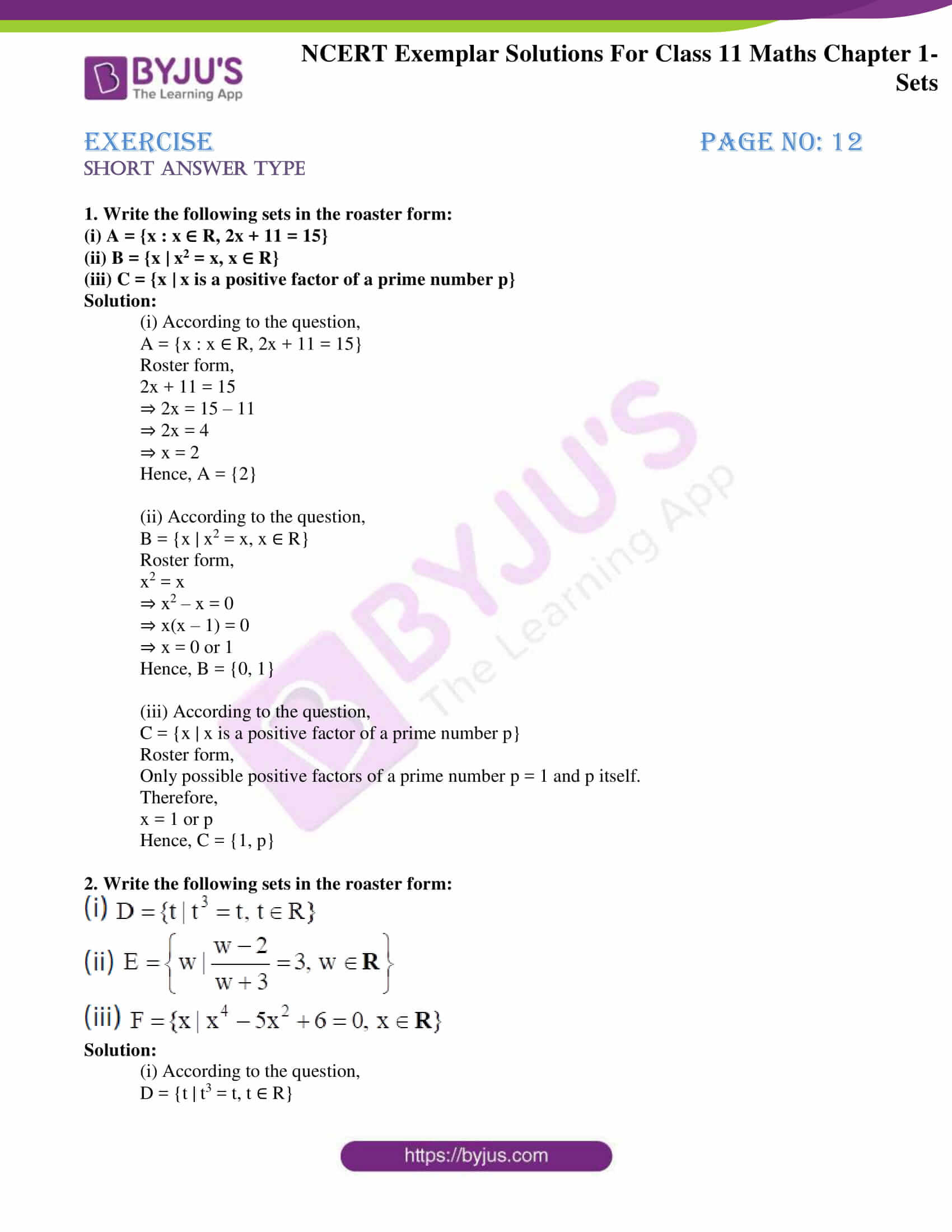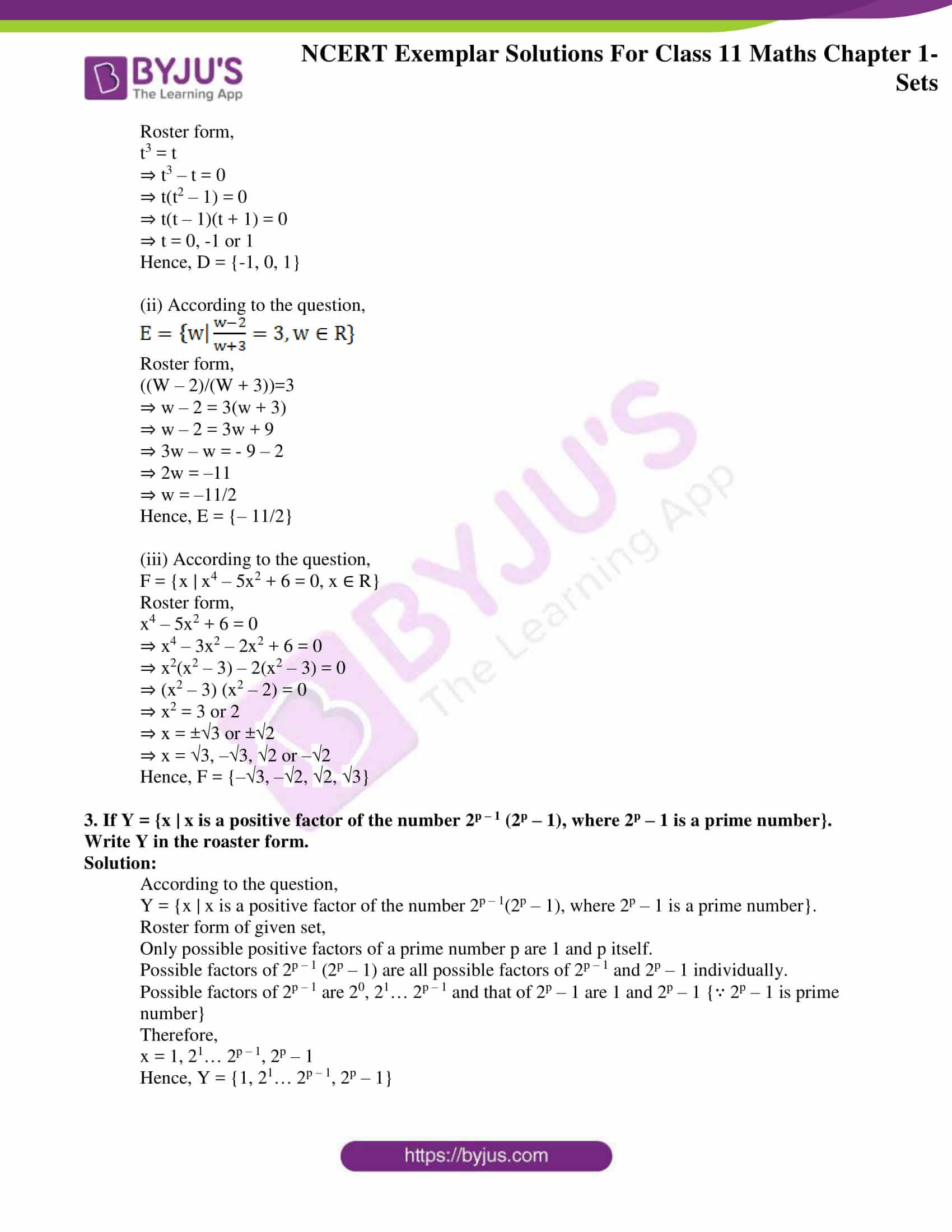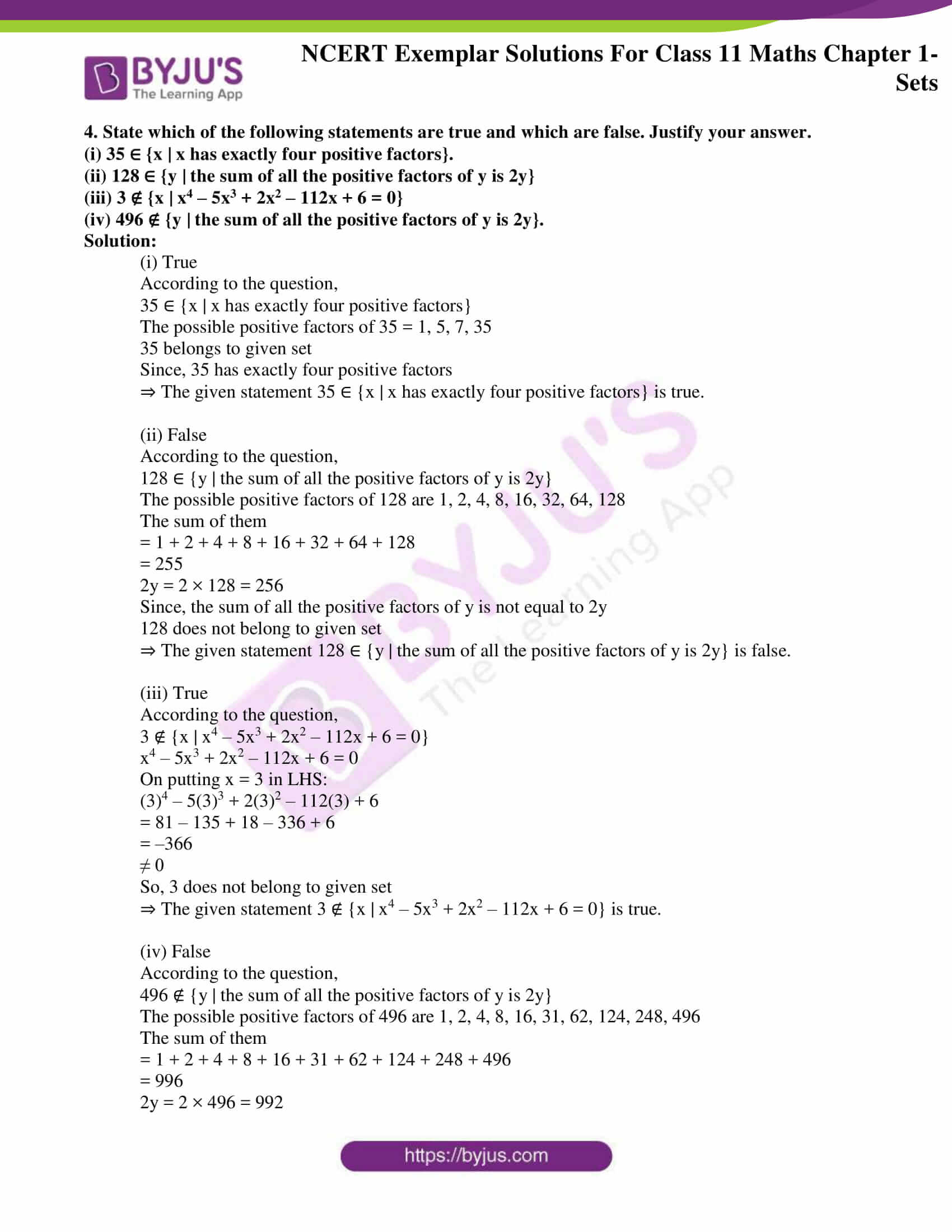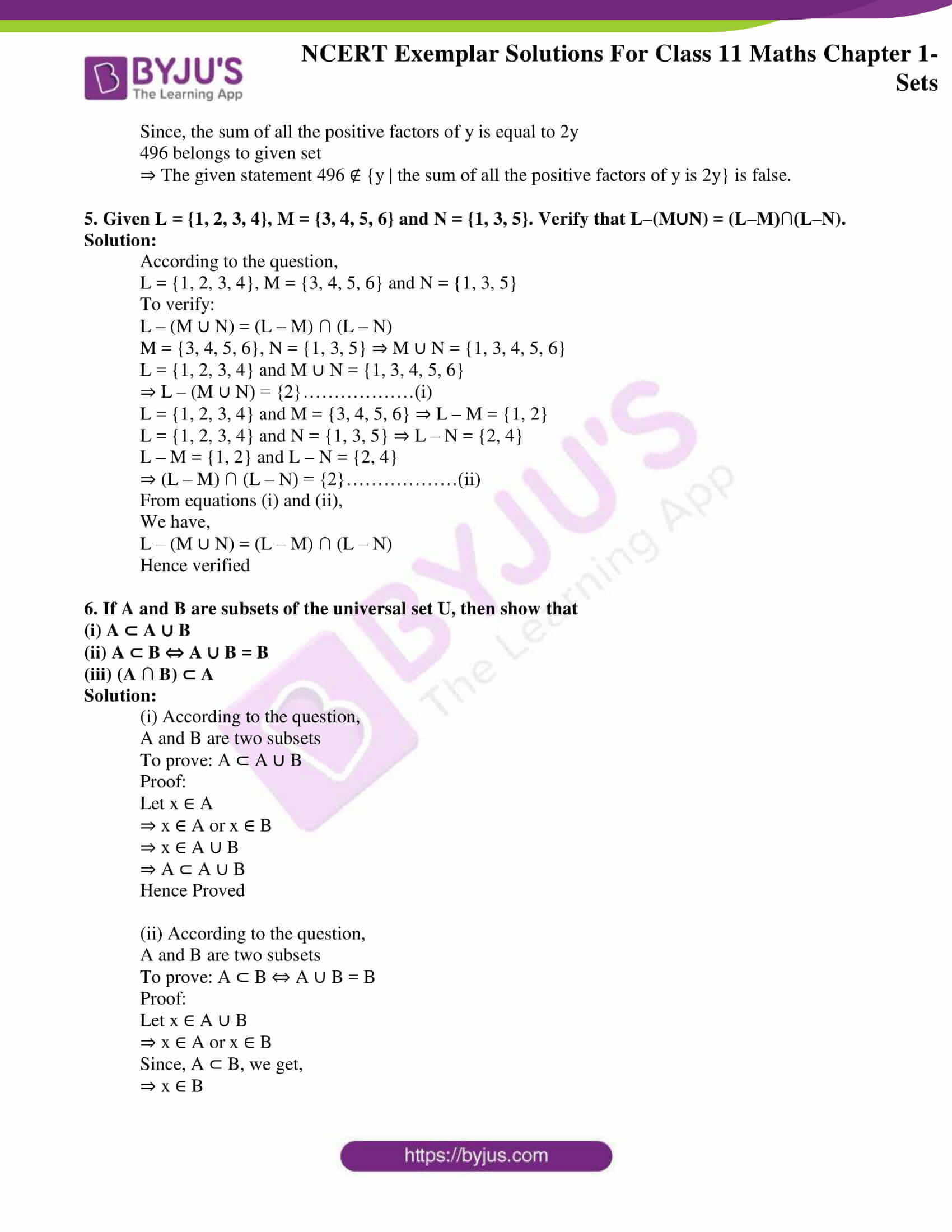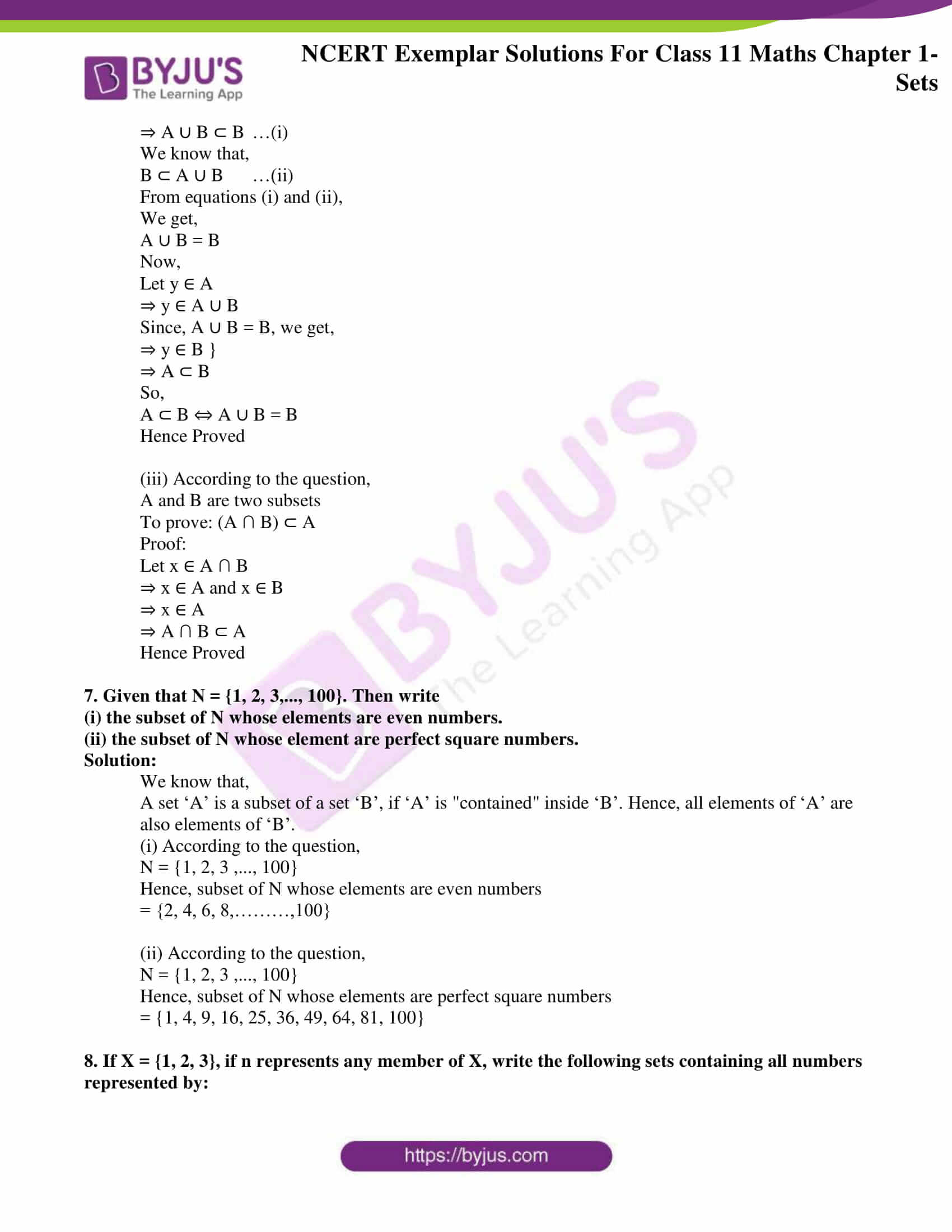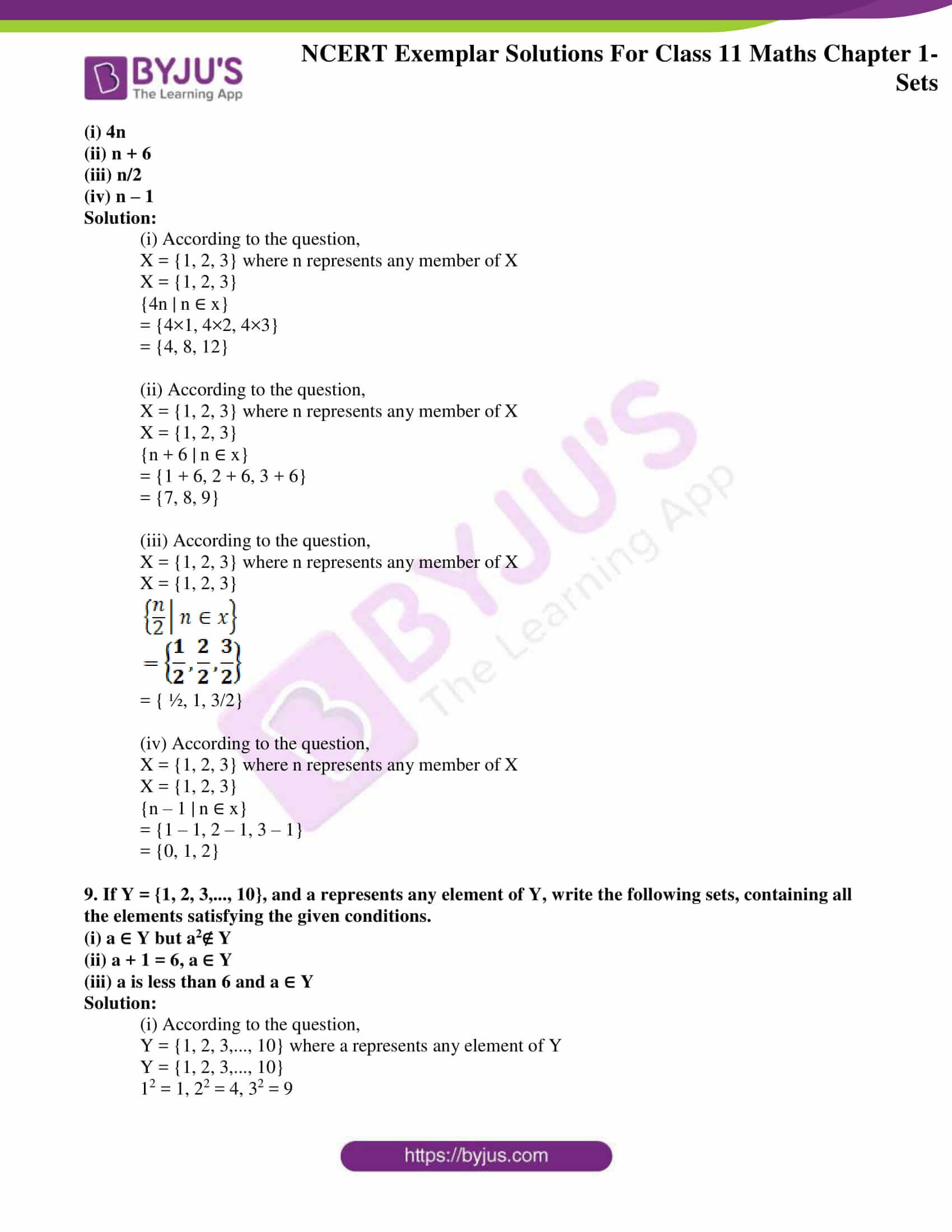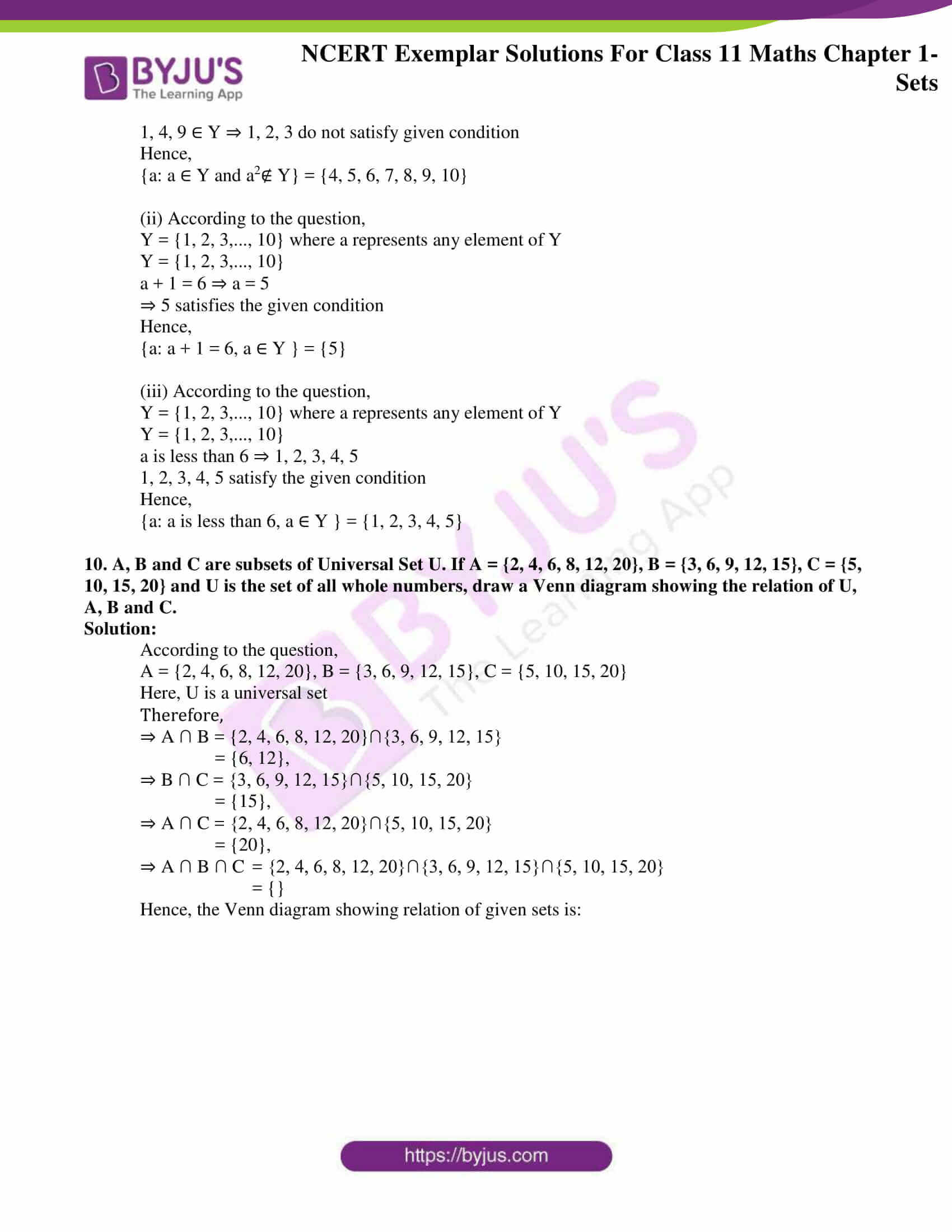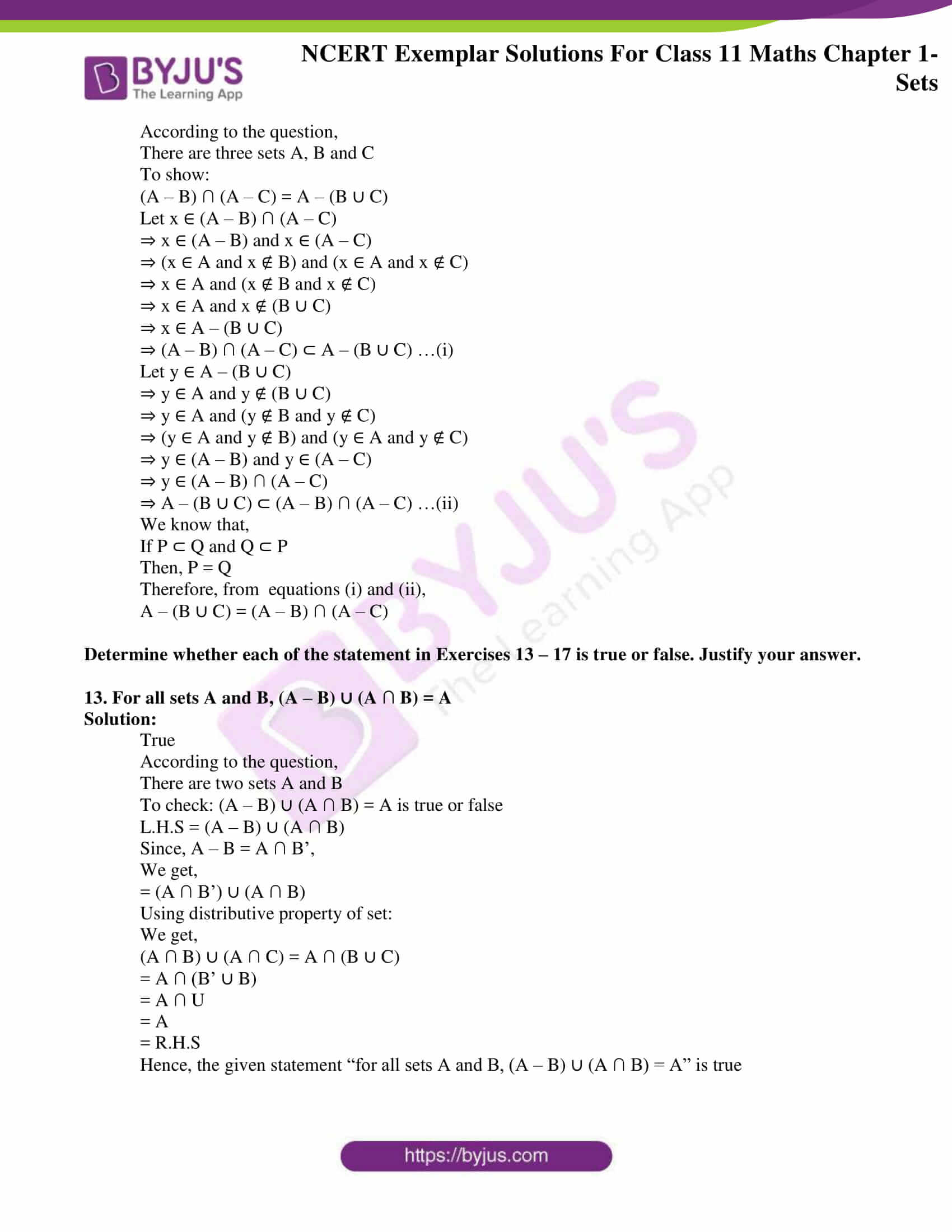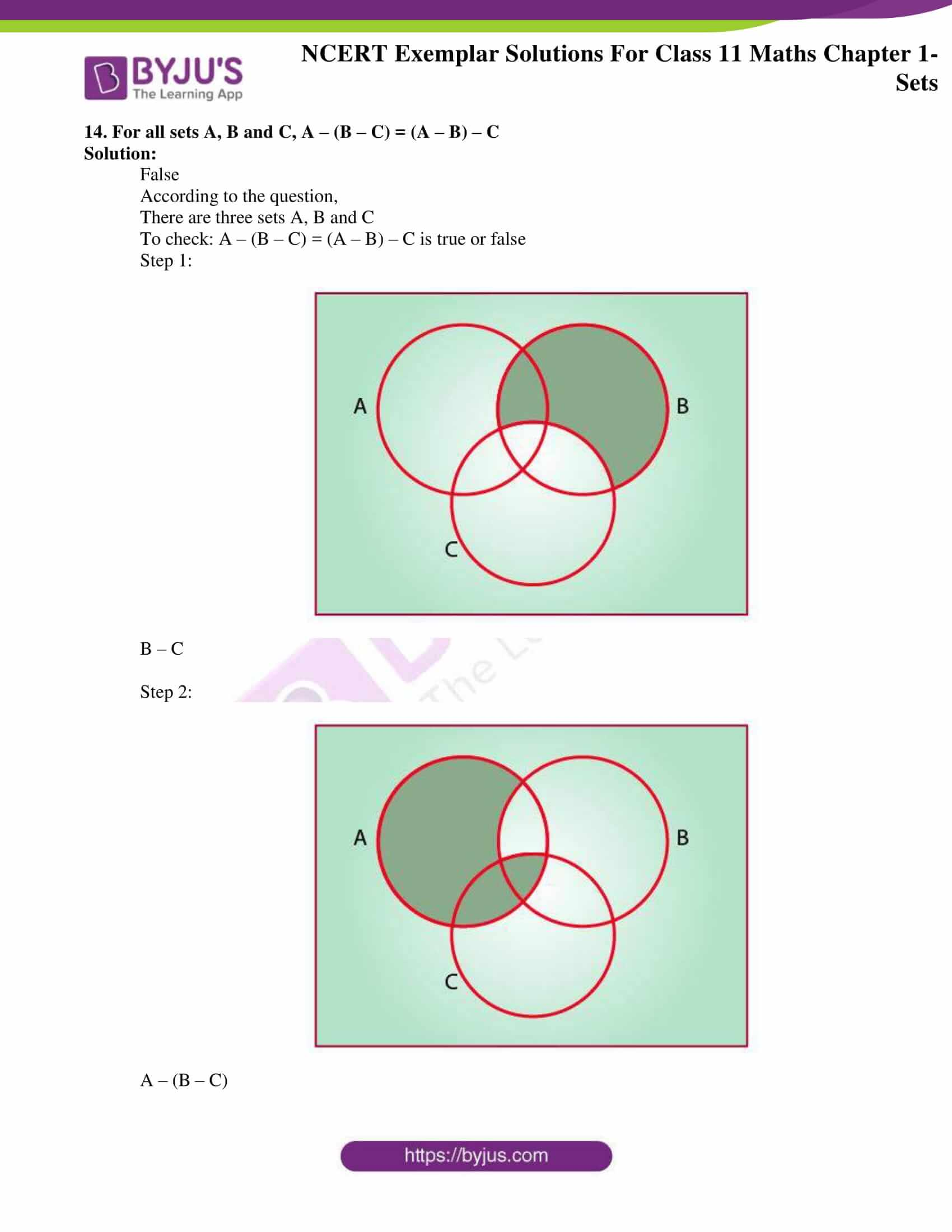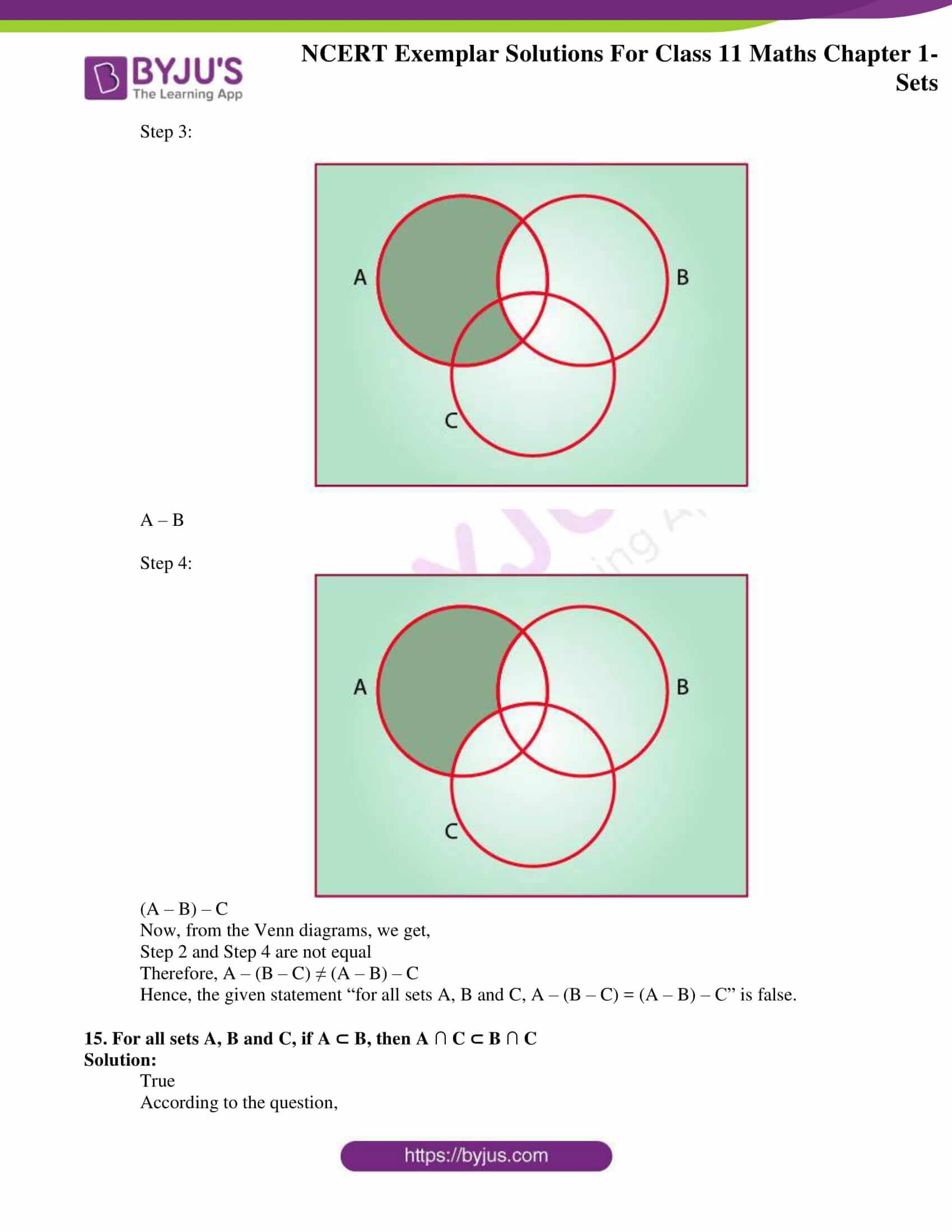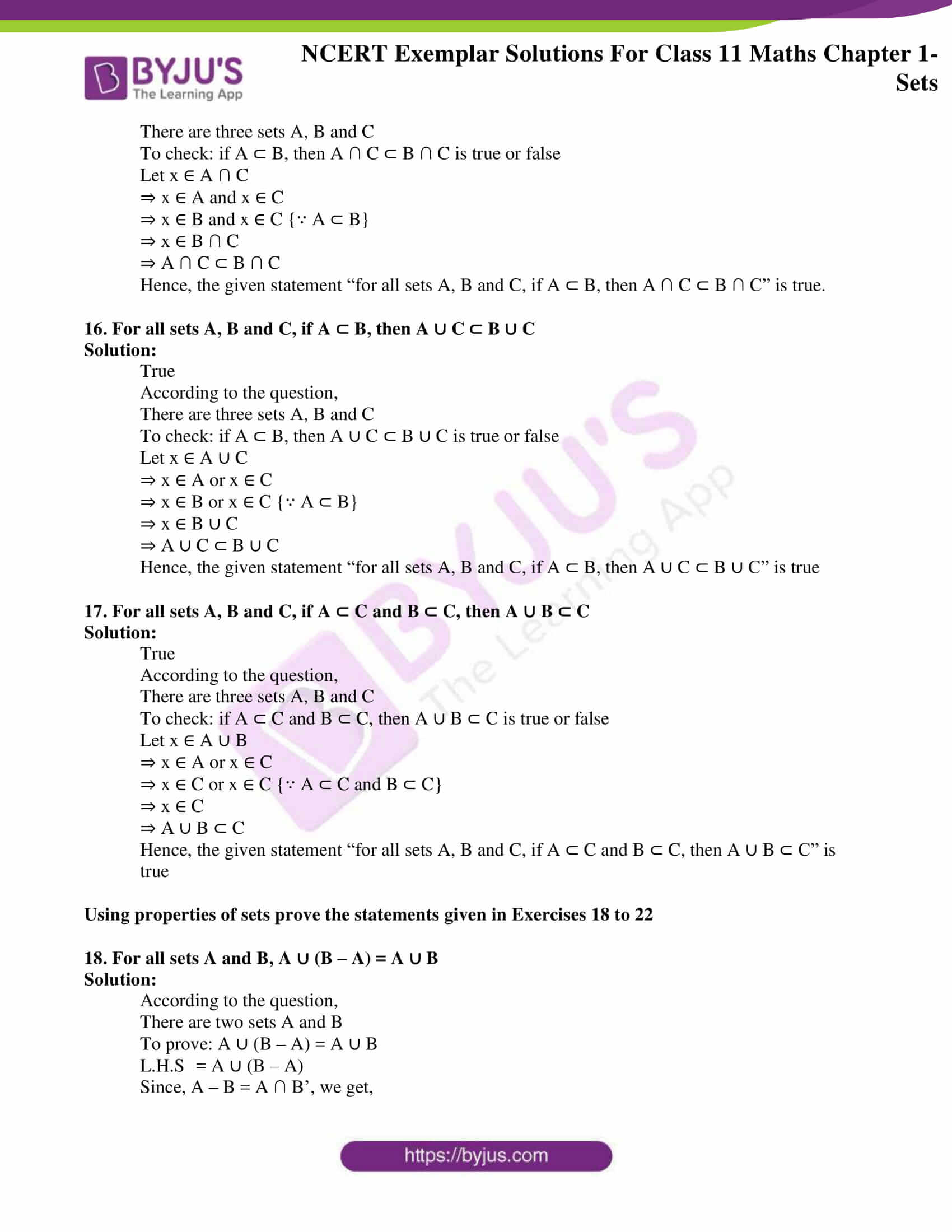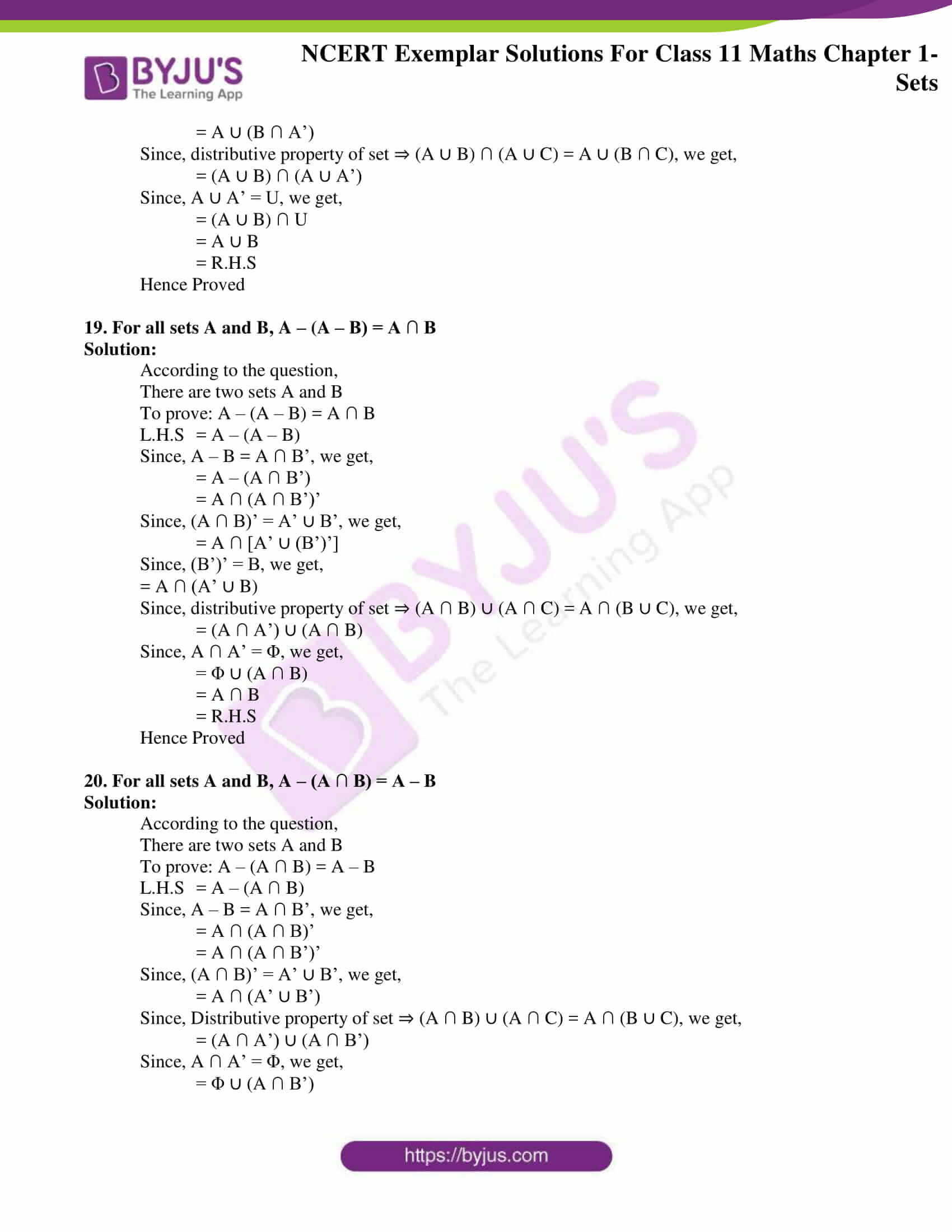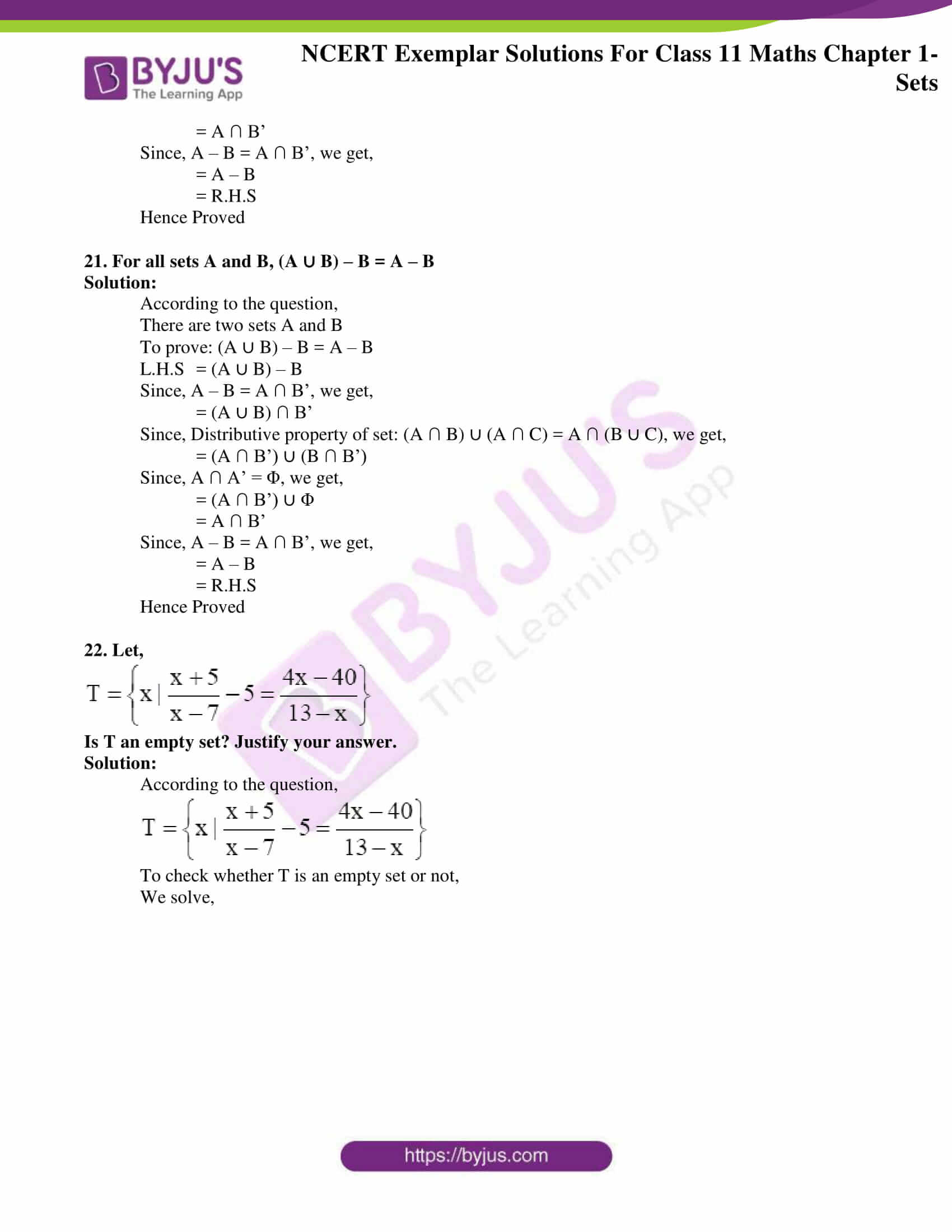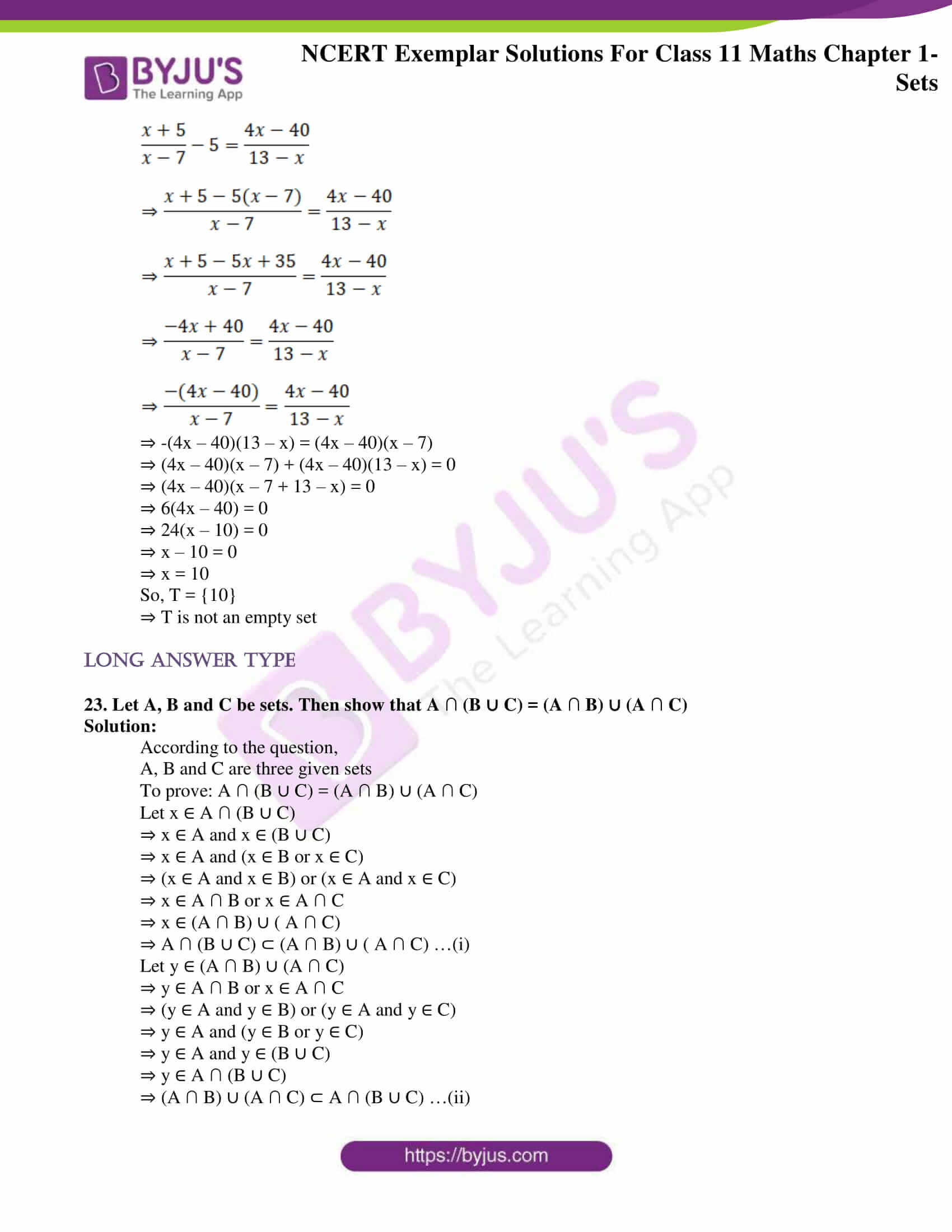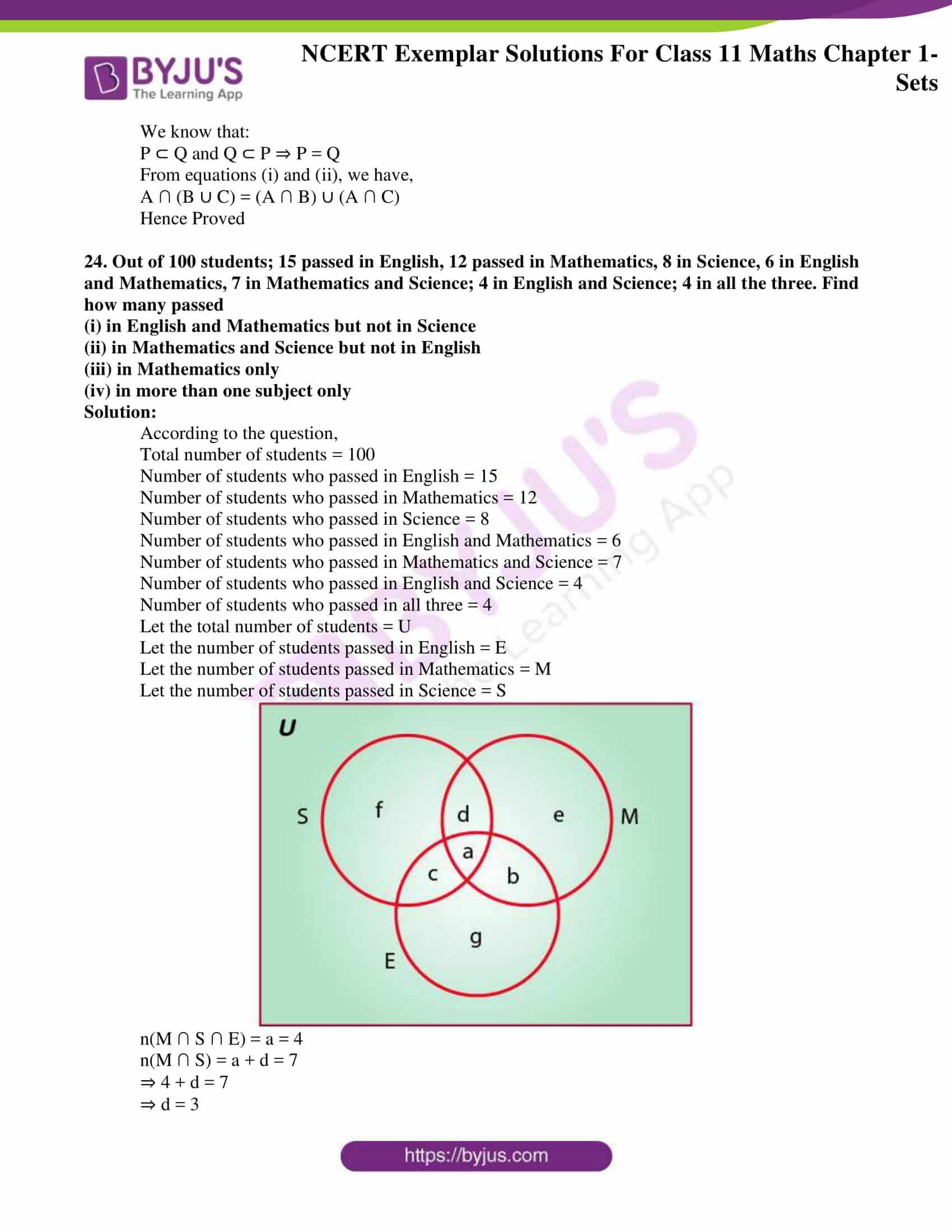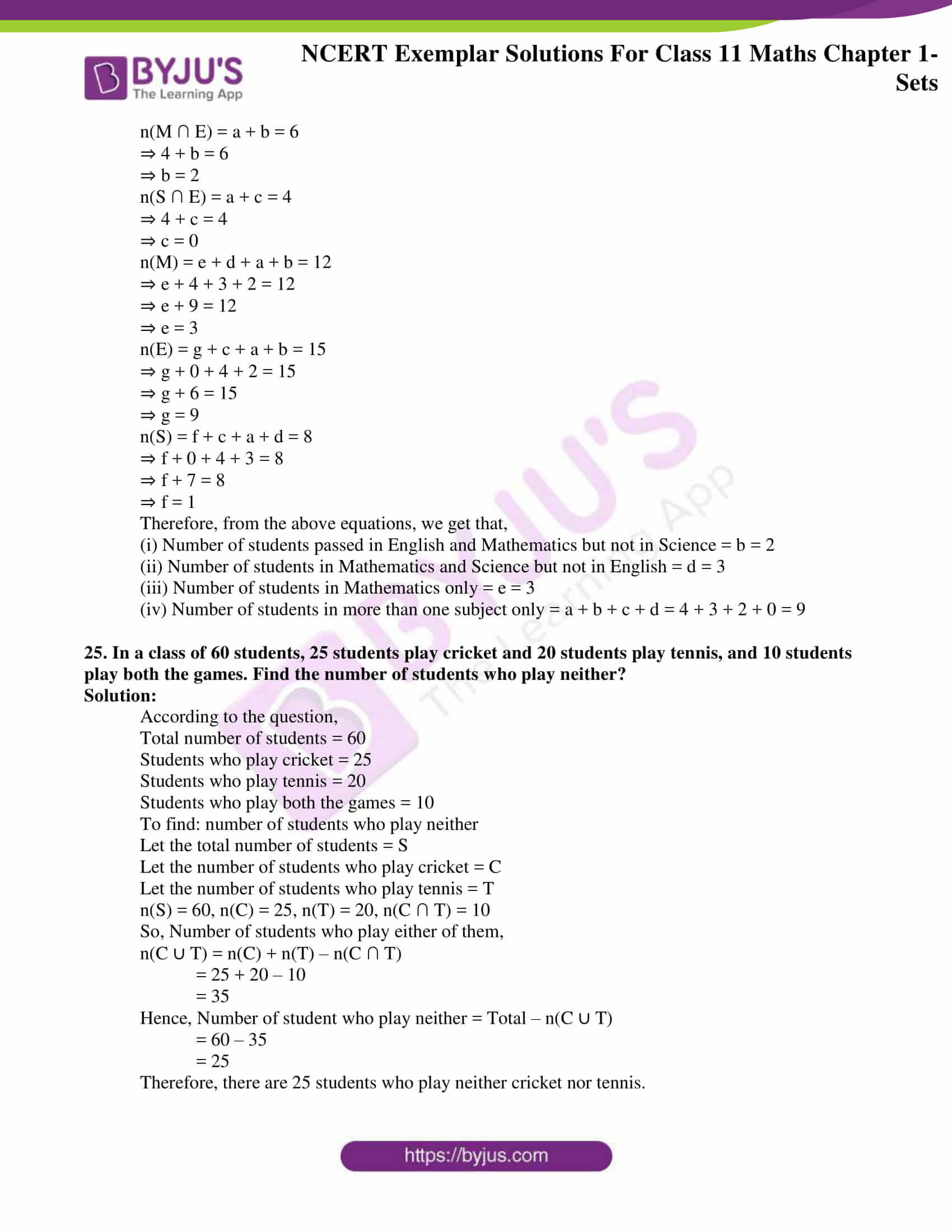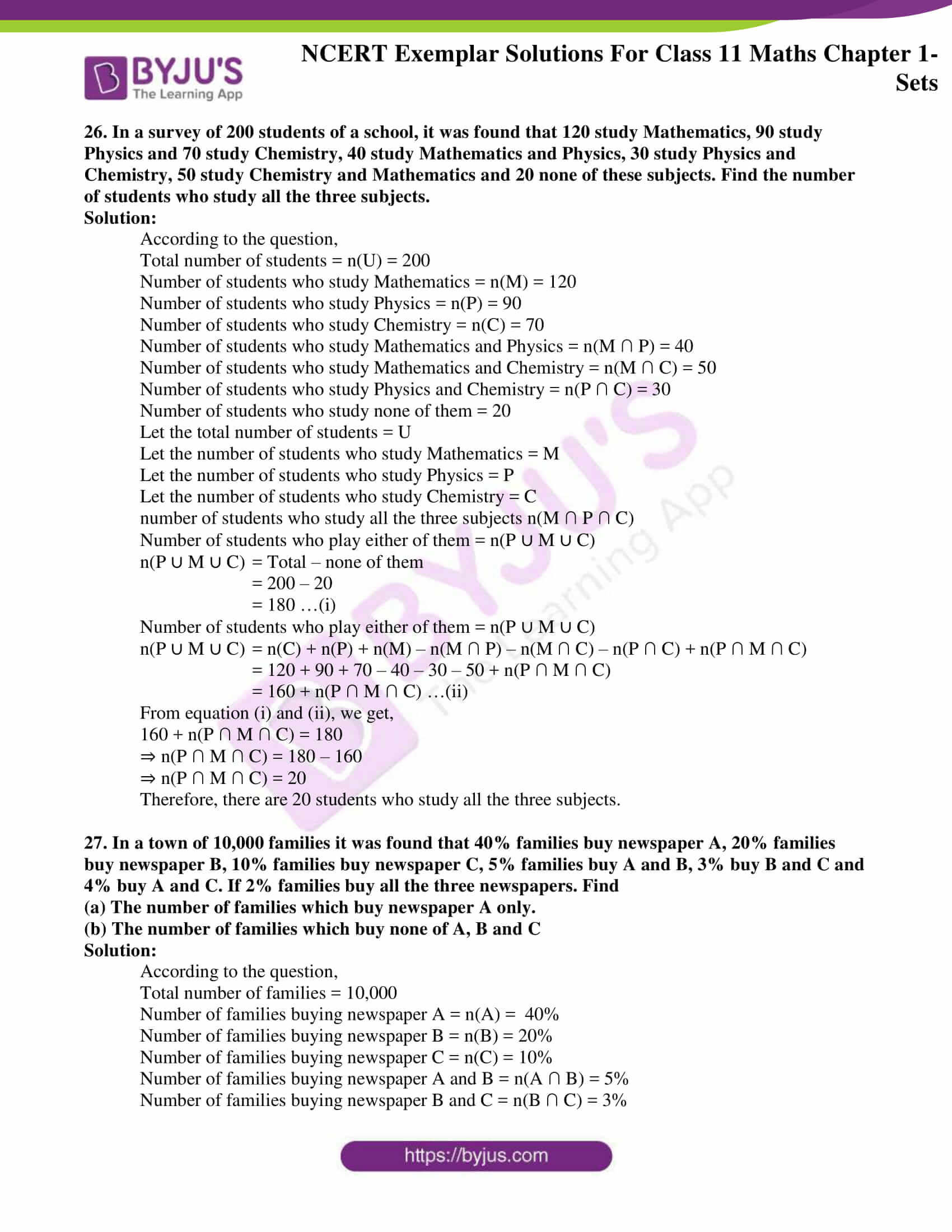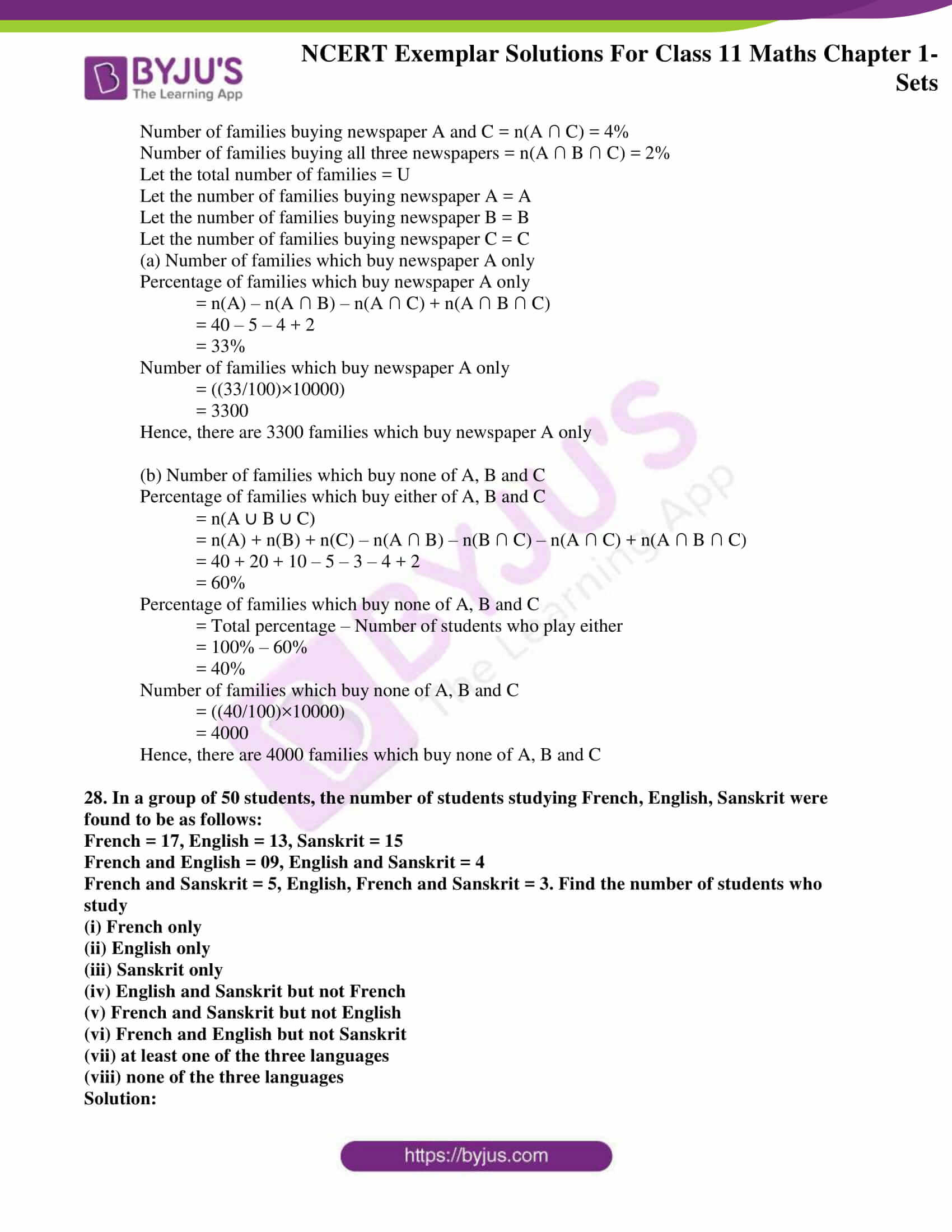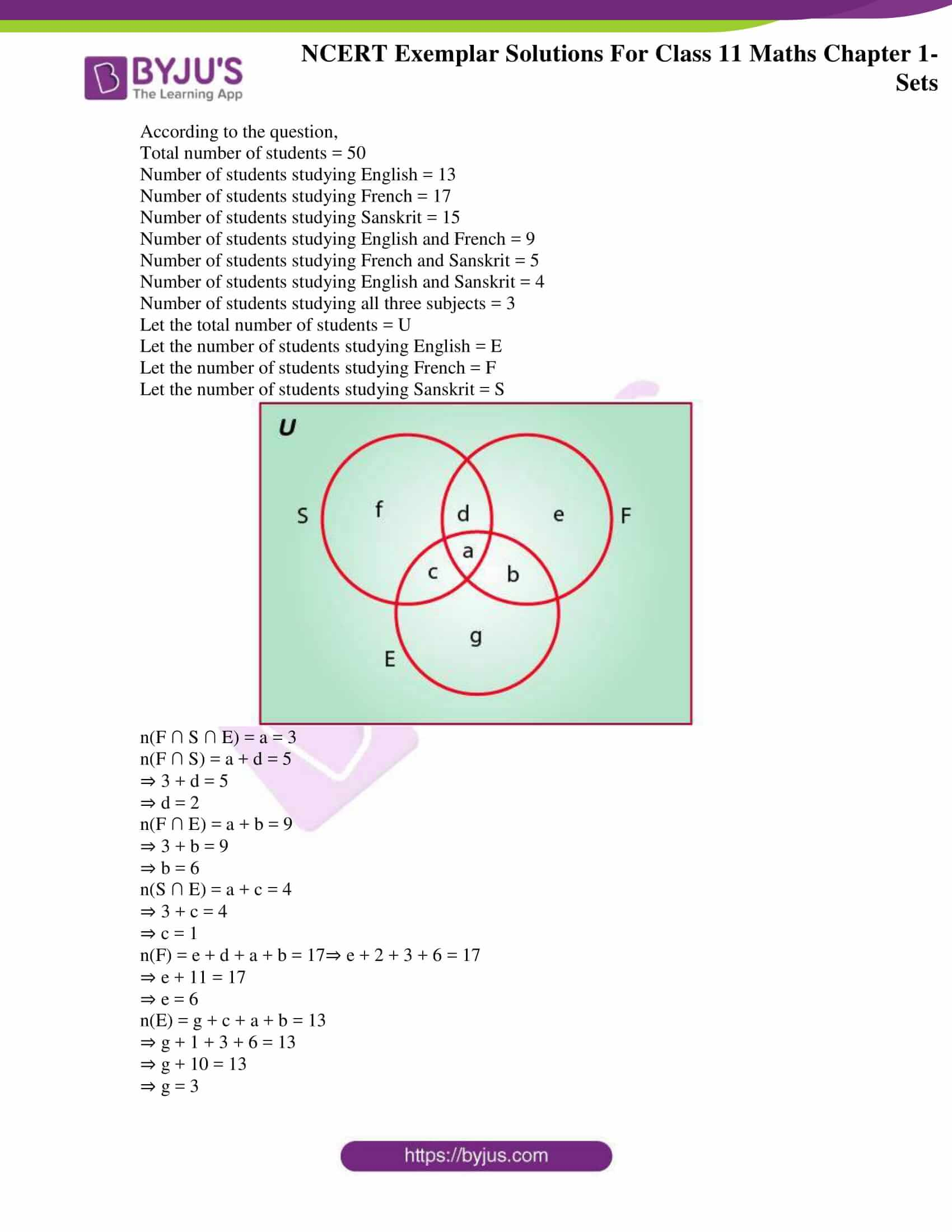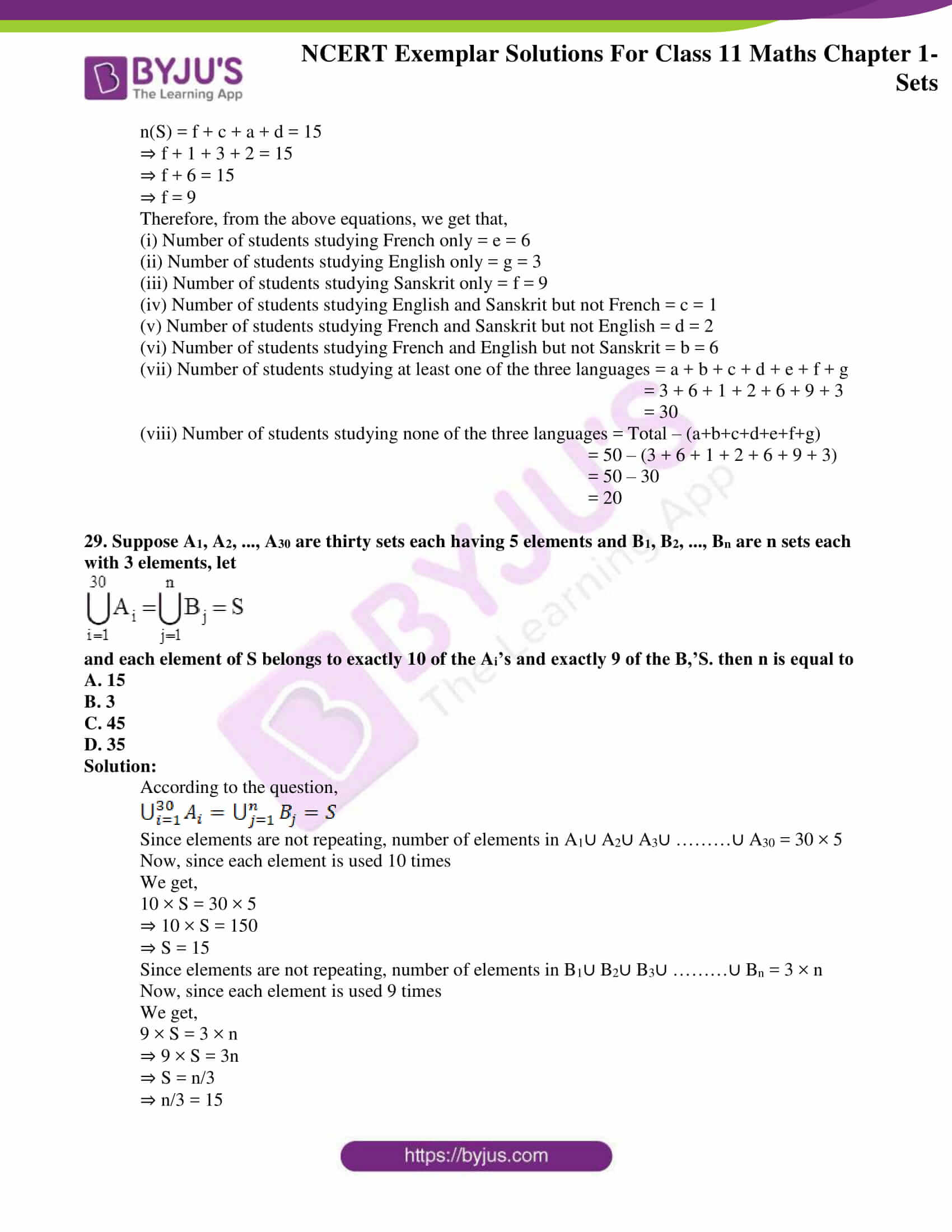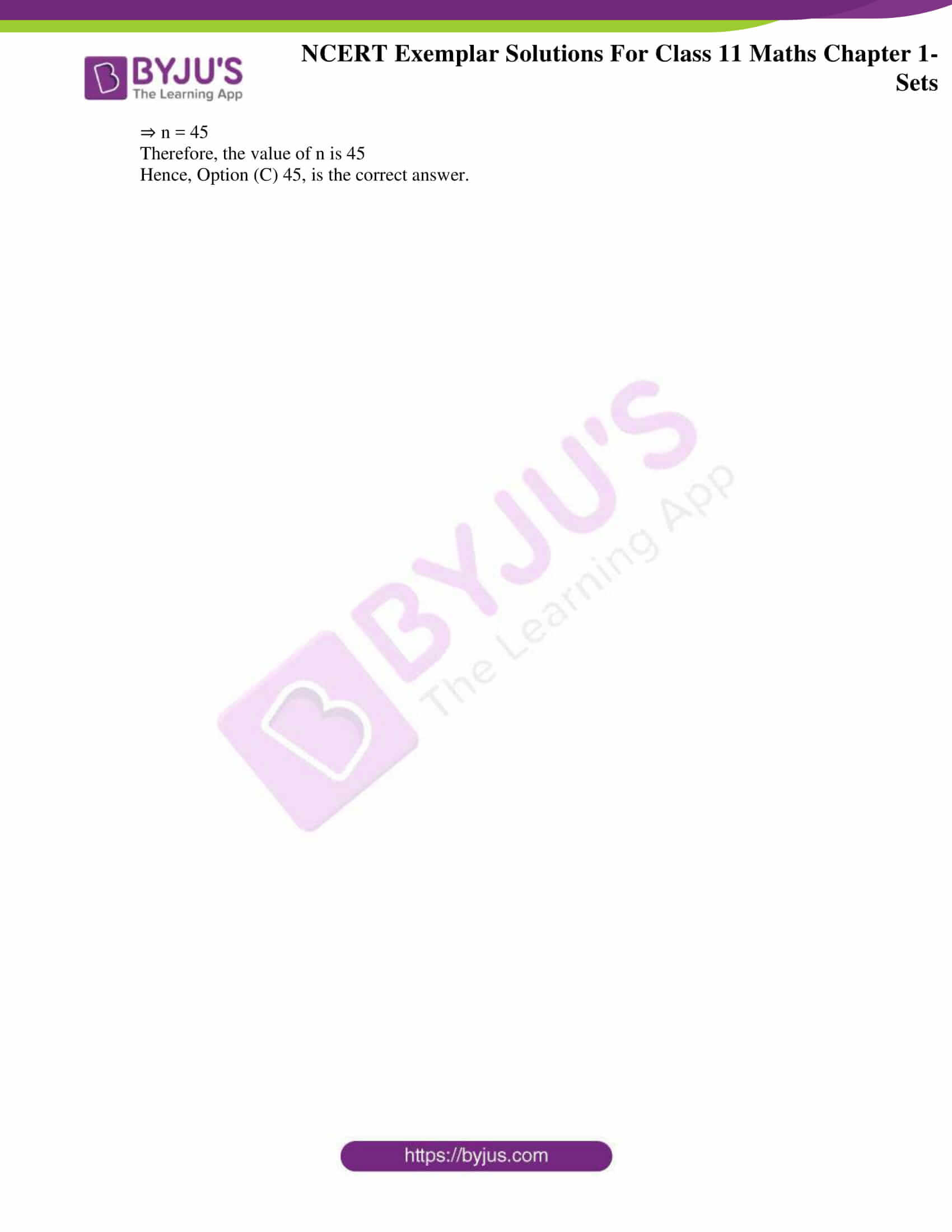### Access Answers to the NCERT Exemplar Class 11 Maths Chapter 1 – Sets

Exercise Page No: 12

1. Write the following sets in the roaster form:

(i) A = {x : x ∈ R, 2x + 11 = 15}
(ii) B = {x | x2 = x, x ∈ R}
(iii) C = {x | x is a positive factor of a prime number p}

Solution:

(i) According to the question,

A = {x : x ∈ R, 2x + 11 = 15}

Roster form,

2x + 11 = 15

⇒ 2x = 15 – 11

⇒ 2x = 4

⇒ x = 2

Hence, A = {2}

(ii) According to the question,

B = {x | x2 = x, x ∈ R}

Roster form,

x2 = x

⇒ x2 – x = 0

⇒ x(x – 1) = 0

⇒ x = 0 or 1

Hence, B = {0, 1}

(iii) According to the question,

C = {x | x is a positive factor of a prime number p}

Roster form,

Only possible positive factors of a prime number p = 1 and p itself.

Therefore,

x = 1 or p

Hence, C = {1, p}

2. Write the following sets in the roaster form: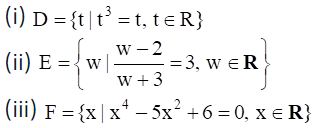Solution:

(i) According to the question,

D = {t | t3 = t, t ∈ R}

Roster form,

t3 = t

⇒ t3 – t = 0

⇒ t(t2 – 1) = 0

⇒ t(t – 1)(t + 1) = 0

⇒ t = 0, -1 or 1

Hence, D = {-1, 0, 1}

(ii) According to the question,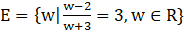Roster form,

((W – 2)/(W + 3))=3

⇒ w – 2 = 3(w + 3)

⇒ w – 2 = 3w + 9

⇒ 3w – w = – 9 – 2

⇒ 2w = –11

⇒ w = –11/2

Hence, E = {– 11/2}

(iii) According to the question,

F = {x | x4 – 5x2 + 6 = 0, x ∈ R}

Roster form,

x4 – 5x2 + 6 = 0

⇒ x4 – 3x2 – 2x2 + 6 = 0

⇒ x2(x2 – 3) – 2(x2 – 3) = 0

⇒ (x2 – 3) (x2 – 2) = 0

⇒ x2 = 3 or 2

⇒ x = ±√3 or ±√2

⇒ x = √3, –√3, √2 or –√2

Hence, F = {–√3, –√2, √2, √3}

3. If Y = {x | x is a positive factor of the number 2p – 1 (2p – 1), where 2p – 1 is a prime number}. Write Y in the roaster form.

Solution:

According to the question,

Y = {x | x is a positive factor of the number 2p – 1(2p – 1), where 2p – 1 is a prime number}.

Roster form of given set,

Only possible positive factors of a prime number p are 1 and p itself.

Possible factors of 2p – 1 (2p – 1) are all possible factors of 2p – 1 and 2p – 1 individually.

Possible factors of 2p – 1 are 20, 21… 2p – 1 and that of 2p – 1 are 1 and 2p – 1 {∵ 2p – 1 is prime number}

Therefore,

x = 1, 21… 2p – 1, 2p – 1

Hence, Y = {1, 21… 2p – 1, 2p – 1}

4. State which of the following statements are true and which are false. Justify your answer.
(i) 35 ∈ {x | x has exactly four positive factors}.
(ii) 128 ∈ {y | the sum of all the positive factors of y is 2y}
(iii) 3 ∉ {x | x4 – 5x3 + 2x2 – 112x + 6 = 0}
(iv) 496 ∉ {y | the sum of all the positive factors of y is 2y}.

Solution:

(i) True

According to the question,

35 ∈ {x | x has exactly four positive factors}

The possible positive factors of 35 = 1, 5, 7, 35

35 belongs to given set

Since, 35 has exactly four positive factors

⇒ The given statement 35 ∈ {x | x has exactly four positive factors} is true.

(ii) False

According to the question,

128 ∈ {y | the sum of all the positive factors of y is 2y}

The possible positive factors of 128 are 1, 2, 4, 8, 16, 32, 64, 128

The sum of them

= 1 + 2 + 4 + 8 + 16 + 32 + 64 + 128

= 255

2y = 2 × 128 = 256

Since, the sum of all the positive factors of y is not equal to 2y

128 does not belong to given set

⇒ The given statement 128 ∈ {y | the sum of all the positive factors of y is 2y} is false.

(iii) True

According to the question,

3 ∉ {x | x4 – 5x3 + 2x2 – 112x + 6 = 0}

x4 – 5x3 + 2x2 – 112x + 6 = 0

On putting x = 3 in LHS:

(3)4 – 5(3)3 + 2(3)2 – 112(3) + 6

= 81 – 135 + 18 – 336 + 6

= –366

≠ 0

So, 3 does not belong to given set

⇒ The given statement 3 ∉ {x | x4 – 5x3 + 2x2 – 112x + 6 = 0} is true.

(iv) False

According to the question,

496 ∉ {y | the sum of all the positive factors of y is 2y}

The possible positive factors of 496 are 1, 2, 4, 8, 16, 31, 62, 124, 248, 496

The sum of them

= 1 + 2 + 4 + 8 + 16 + 31 + 62 + 124 + 248 + 496

= 996

2y = 2 × 496 = 992

Since, the sum of all the positive factors of y is equal to 2y

496 belongs to given set

⇒ The given statement 496 ∉ {y | the sum of all the positive factors of y is 2y} is false.

5. Given L = {1, 2, 3, 4}, M = {3, 4, 5, 6} and N = {1, 3, 5}. Verify that L–(M∪N) = (L–M)∩(L–N).

Solution:

According to the question,

L = {1, 2, 3, 4}, M = {3, 4, 5, 6} and N = {1, 3, 5}

To verify:

L – (M ∪ N) = (L – M) ∩ (L – N)

M = {3, 4, 5, 6}, N = {1, 3, 5} ⇒ M ∪ N = {1, 3, 4, 5, 6}

L = {1, 2, 3, 4} and M ∪ N = {1, 3, 4, 5, 6}

⇒ L – (M ∪ N) = {2}………………(i)

L = {1, 2, 3, 4} and M = {3, 4, 5, 6} ⇒ L – M = {1, 2}

L = {1, 2, 3, 4} and N = {1, 3, 5} ⇒ L – N = {2, 4}

L – M = {1, 2} and L – N = {2, 4}

⇒ (L – M) ∩ (L – N) = {2}………………(ii)

From equations (i) and (ii),

We have,

L – (M ∪ N) = (L – M) ∩ (L – N)

Hence verified

6. If A and B are subsets of the universal set U, then show that
(i) A ⊂ A ∪ B
(ii) A ⊂ B ⇔ A ∪ B = B
(iii) (A ∩ B) ⊂ A

Solution:

(i) According to the question,

A and B are two subsets

To prove: A ⊂ A ∪ B

Proof:

Let x ∈ A

⇒ x ∈ A or x ∈ B

⇒ x ∈ A ∪ B

⇒ A ⊂ A ∪ B

Hence Proved

(ii) According to the question,

A and B are two subsets

To prove: A ⊂ B ⇔ A ∪ B = B

Proof:

Let x ∈ A ∪ B

⇒ x ∈ A or x ∈ B

Since, A ⊂ B, we get,

⇒ x ∈ B

⇒ A ∪ B ⊂ B …(i)

We know that,

B ⊂ A ∪ B …(ii)

From equations (i) and (ii),

We get,

A ∪ B = B

Now,

Let y ∈ A

⇒ y ∈ A ∪ B

Since, A ∪ B = B, we get,

⇒ y ∈ B }

⇒ A ⊂ B

So,

A ⊂ B ⇔ A ∪ B = B

Hence Proved

(iii) According to the question,

A and B are two subsets

To prove: (A ∩ B) ⊂ A

Proof:

Let x ∈ A ∩ B

⇒ x ∈ A and x ∈ B

⇒ x ∈ A

⇒ A ∩ B ⊂ A

Hence Proved

7. Given that N = {1, 2, 3,…, 100}. Then write

(i) the subset of N whose elements are even numbers.
(ii) the subset of N whose element are perfect square numbers.

Solution:

We know that,

A set ‘A’ is a subset of a set ‘B’, if ‘A’ is “contained” inside ‘B’. Hence, all elements of ‘A’ are also elements of ‘B’.

(i) According to the question,

N = {1, 2, 3 ,…, 100}

Hence, subset of N whose elements are even numbers

= {2, 4, 6, 8,………,100}

(ii) According to the question,

N = {1, 2, 3 ,…, 100}

Hence, subset of N whose elements are perfect square numbers

= {1, 4, 9, 16, 25, 36, 49, 64, 81, 100}

8. If X = {1, 2, 3}, if n represents any member of X, write the following sets containing all numbers represented by:

(i) 4n
(ii) n + 6
(iii) n/2
(iv) n – 1

Solution:

(i) According to the question,

X = {1, 2, 3} where n represents any member of X

X = {1, 2, 3}

{4n | n ∈ x}

= {4×1, 4×2, 4×3}

= {4, 8, 12}

(ii) According to the question,

X = {1, 2, 3} where n represents any member of X

X = {1, 2, 3}

{n + 6 | n ∈ x}

= {1 + 6, 2 + 6, 3 + 6}

= {7, 8, 9}

(iii) According to the question,

X = {1, 2, 3} where n represents any member of X

X = {1, 2, 3}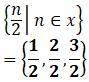= { ½, 1, 3/2}

(iv) According to the question,

X = {1, 2, 3} where n represents any member of X

X = {1, 2, 3}

{n – 1 | n ∈ x}

= {1 – 1, 2 – 1, 3 – 1}

= {0, 1, 2}

9. If Y = {1, 2, 3,…, 10}, and a represents any element of Y, write the following sets, containing all the elements satisfying the given conditions.
(i) a ∈ Y but a2∉ Y
(ii) a + 1 = 6, a ∈ Y
(iii) a is less than 6 and a ∈ Y

Solution:

(i) According to the question,

Y = {1, 2, 3,…, 10} where a represents any element of Y

Y = {1, 2, 3,…, 10}

12 = 1, 22 = 4, 32 = 9

1, 4, 9 ∈ Y ⇒ 1, 2, 3 do not satisfy given condition

Hence,

{a: a ∈ Y and a2∉ Y} = {4, 5, 6, 7, 8, 9, 10}

(ii) According to the question,

Y = {1, 2, 3,…, 10} where a represents any element of Y

Y = {1, 2, 3,…, 10}

a + 1 = 6 ⇒ a = 5

⇒ 5 satisfies the given condition

Hence,

{a: a + 1 = 6, a ∈ Y } = {5}

(iii) According to the question,

Y = {1, 2, 3,…, 10} where a represents any element of Y

Y = {1, 2, 3,…, 10}

a is less than 6 ⇒ 1, 2, 3, 4, 5

1, 2, 3, 4, 5 satisfy the given condition

Hence,

{a: a is less than 6, a ∈ Y } = {1, 2, 3, 4, 5}

10. A, B and C are subsets of Universal Set U. If A = {2, 4, 6, 8, 12, 20}, B = {3, 6, 9, 12, 15}, C = {5, 10, 15, 20} and U is the set of all whole numbers, draw a Venn diagram showing the relation of U, A, B and C.

Solution:

According to the question,

A = {2, 4, 6, 8, 12, 20}, B = {3, 6, 9, 12, 15}, C = {5, 10, 15, 20}

Here, U is a universal set

Therefore,

⇒ A ∩ B = {2, 4, 6, 8, 12, 20}∩{3, 6, 9, 12, 15}

= {6, 12},

⇒ B ∩ C = {3, 6, 9, 12, 15}∩{5, 10, 15, 20}

= {15},

⇒ A ∩ C = {2, 4, 6, 8, 12, 20}∩{5, 10, 15, 20}

= {20},

⇒ A ∩ B ∩ C = {2, 4, 6, 8, 12, 20}∩{3, 6, 9, 12, 15}∩{5, 10, 15, 20}

= {}

Hence, the Venn diagram showing relation of given sets is: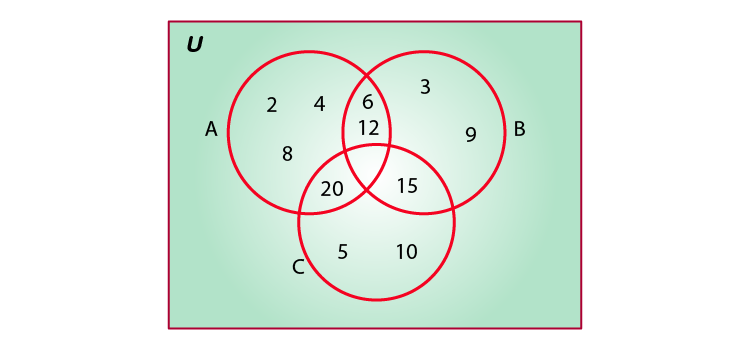11. Let U be the set of all boys and girls in a school, G be the set of all girls in the school, B be the set of all boys in the school, and S be the set of all students in the school who take swimming. Some, but not all, students in the school take swimming. Draw a Venn diagram showing one of the possible interrelationship among sets U, G, B and S.

Solution:

According to the question,

There are four sets U, G, B, S

Here,

U = a universal set containing set of all boys and girls in a school

G = set of all girls in the school

B = set of all boys in the school

S = set of all students in the school who take swimming

The Venn diagram showing relation of given sets is: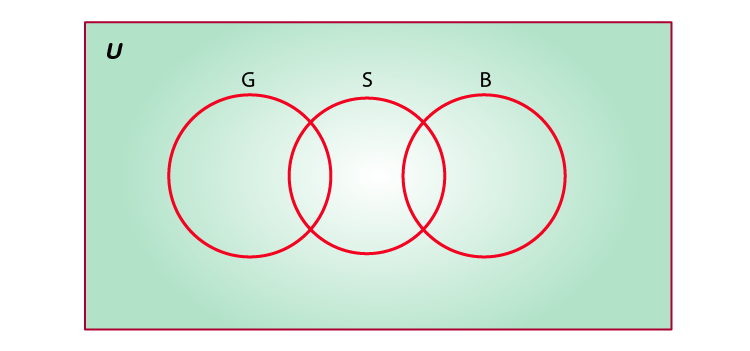12. For all sets A, B and C, show that (A – B) ∩ (A – C) = A – (B ∪ C)

Solution:

According to the question,

There are three sets A, B and C

To show:

(A – B) ∩ (A – C) = A – (B ∪ C)

Let x ∈ (A – B) ∩ (A – C)

⇒ x ∈ (A – B) and x ∈ (A – C)

⇒ (x ∈ A and x ∉ B) and (x ∈ A and x ∉ C)

⇒ x ∈ A and (x ∉ B and x ∉ C)

⇒ x ∈ A and x ∉ (B ∪ C)

⇒ x ∈ A – (B ∪ C)

⇒ (A – B) ∩ (A – C) ⊂ A – (B ∪ C) …(i)

Let y ∈ A – (B ∪ C)

⇒ y ∈ A and y ∉ (B ∪ C)

⇒ y ∈ A and (y ∉ B and y ∉ C)

⇒ (y ∈ A and y ∉ B) and (y ∈ A and y ∉ C)

⇒ y ∈ (A – B) and y ∈ (A – C)

⇒ y ∈ (A – B) ∩ (A – C)

⇒ A – (B ∪ C) ⊂ (A – B) ∩ (A – C) …(ii)

We know that,

If P ⊂ Q and Q ⊂ P

Then, P = Q

Therefore, from equations (i) and (ii),

A – (B ∪ C) = (A – B) ∩ (A – C)

Determine whether each of the statement in Exercises 13 – 17 is true or false. Justify your answer.

13. For all sets A and B, (A – B) ∪ (A ∩ B) = A

Solution:

True

According to the question,

There are two sets A and B

To check: (A – B) ∪ (A ∩ B) = A is true or false

L.H.S = (A – B) ∪ (A ∩ B)

Since, A – B = A ∩ B’,

We get,

= (A ∩ B’) ∪ (A ∩ B)

Using distributive property of set:

We get,

(A ∩ B) ∪ (A ∩ C) = A ∩ (B ∪ C)

= A ∩ (B’ ∪ B)

= A ∩ U

= A

= R.H.S

Hence, the given statement “for all sets A and B, (A – B) ∪ (A ∩ B) = A” is true

14. For all sets A, B and C, A – (B – C) = (A – B) – C

Solution:

False

According to the question,

There are three sets A, B and C

To check: A – (B – C) = (A – B) – C is true or false

Step 1: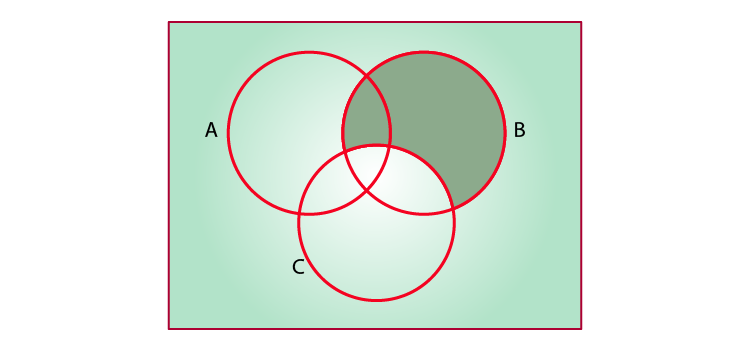B – C

Step 2: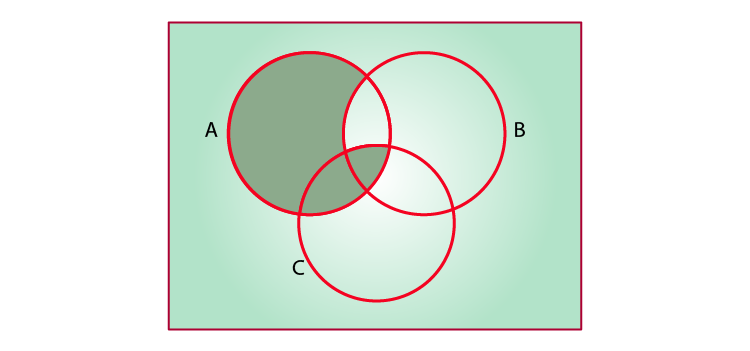A – (B – C)

Step 3: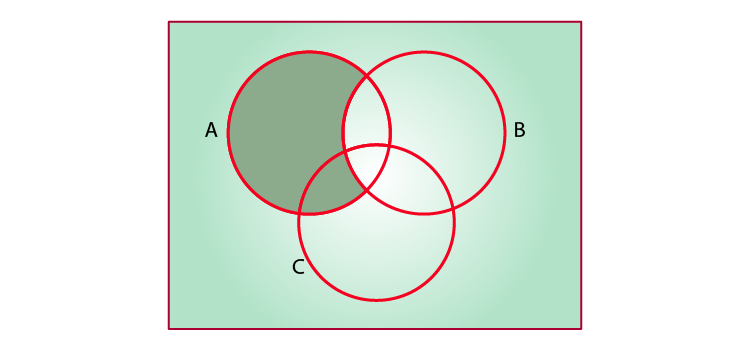A – B

Step 4: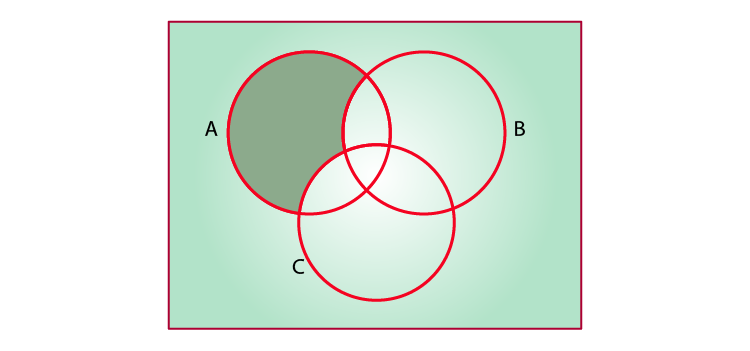(A – B) – C

Now, from the Venn diagrams, we get,

Step 2 and Step 4 are not equal

Therefore, A – (B – C) ≠ (A – B) – C

Hence, the given statement “for all sets A, B and C, A – (B – C) = (A – B) – C” is false.

15. For all sets A, B and C, if A ⊂ B, then A ∩ C ⊂ B ∩ C

Solution:

True

According to the question,

There are three sets A, B and C

To check: if A ⊂ B, then A ∩ C ⊂ B ∩ C is true or false

Let x ∈ A ∩ C

⇒ x ∈ A and x ∈ C

⇒ x ∈ B and x ∈ C {∵ A ⊂ B}

⇒ x ∈ B ∩ C

⇒ A ∩ C ⊂ B ∩ C

Hence, the given statement “for all sets A, B and C, if A ⊂ B, then A ∩ C ⊂ B ∩ C” is true.

16. For all sets A, B and C, if A ⊂ B, then A ∪ C ⊂ B ∪ C

Solution:

True

According to the question,

There are three sets A, B and C

To check: if A ⊂ B, then A ∪ C ⊂ B ∪ C is true or false

Let x ∈ A ∪ C

⇒ x ∈ A or x ∈ C

⇒ x ∈ B or x ∈ C {∵ A ⊂ B}

⇒ x ∈ B ∪ C

⇒ A ∪ C ⊂ B ∪ C

Hence, the given statement “for all sets A, B and C, if A ⊂ B, then A ∪ C ⊂ B ∪ C” is true

17. For all sets A, B and C, if A ⊂ C and B ⊂ C, then A ∪ B ⊂ C

Solution:

True

According to the question,

There are three sets A, B and C

To check: if A ⊂ C and B ⊂ C, then A ∪ B ⊂ C is true or false

Let x ∈ A ∪ B

⇒ x ∈ A or x ∈ C

⇒ x ∈ C or x ∈ C {∵ A ⊂ C and B ⊂ C}

⇒ x ∈ C

⇒ A ∪ B ⊂ C

Hence, the given statement “for all sets A, B and C, if A ⊂ C and B ⊂ C, then A ∪ B ⊂ C” is true

Using properties of sets prove the statements given in Exercises 18 to 22

18. For all sets A and B, A ∪ (B – A) = A ∪ B

Solution:

According to the question,

There are two sets A and B

To prove: A ∪ (B – A) = A ∪ B

L.H.S = A ∪ (B – A)

Since, A – B = A ∩ B’, we get,

= A ∪ (B ∩ A’)

Since, distributive property of set ⇒ (A ∪ B) ∩ (A ∪ C) = A ∪ (B ∩ C), we get,

= (A ∪ B) ∩ (A ∪ A’)

Since, A ∪ A’ = U, we get,

= (A ∪ B) ∩ U

= A ∪ B

= R.H.S

Hence Proved

19. For all sets A and B, A – (A – B) = A ∩ B

Solution:

According to the question,

There are two sets A and B

To prove: A – (A – B) = A ∩ B

L.H.S = A – (A – B)

Since, A – B = A ∩ B’, we get,

= A – (A ∩ B’)

= A ∩ (A ∩ B’)’

Since, (A ∩ B)’ = A’ ∪ B’, we get,

= A ∩ [A’ ∪ (B’)’]

Since, (B’)’ = B, we get,

= A ∩ (A’ ∪ B)

Since, distributive property of set ⇒ (A ∩ B) ∪ (A ∩ C) = A ∩ (B ∪ C), we get,

= (A ∩ A’) ∪ (A ∩ B)

Since, A ∩ A’ = Φ, we get,

= Φ ∪ (A ∩ B)

= A ∩ B

= R.H.S

Hence Proved

20. For all sets A and B, A – (A ∩ B) = A – B

Solution:

According to the question,

There are two sets A and B

To prove: A – (A ∩ B) = A – B

L.H.S = A – (A ∩ B)

Since, A – B = A ∩ B’, we get,

= A ∩ (A ∩ B)’

= A ∩ (A ∩ B’)’

Since, (A ∩ B)’ = A’ ∪ B’, we get,

= A ∩ (A’ ∪ B’)

Since, Distributive property of set ⇒ (A ∩ B) ∪ (A ∩ C) = A ∩ (B ∪ C), we get,

= (A ∩ A’) ∪ (A ∩ B’)

Since, A ∩ A’ = Φ, we get,

= Φ ∪ (A ∩ B’)

= A ∩ B’

Since, A – B = A ∩ B’, we get,

= A – B

= R.H.S

Hence Proved

21. For all sets A and B, (A ∪ B) – B = A – B

Solution:

According to the question,

There are two sets A and B

To prove: (A ∪ B) – B = A – B

L.H.S = (A ∪ B) – B

Since, A – B = A ∩ B’, we get,

= (A ∪ B) ∩ B’

Since, Distributive property of set: (A ∩ B) ∪ (A ∩ C) = A ∩ (B ∪ C), we get,

= (A ∩ B’) ∪ (B ∩ B’)

Since, A ∩ A’ = Φ, we get,

= (A ∩ B’) ∪ Φ

= A ∩ B’

Since, A – B = A ∩ B’, we get,

= A – B

= R.H.S

Hence Proved

22. Let,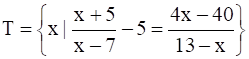Solution:

According to the question,To check whether T is an empty set or not,

We solve,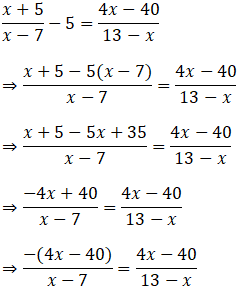⇒ -(4x – 40)(13 – x) = (4x – 40)(x – 7)

⇒ (4x – 40)(x – 7) + (4x – 40)(13 – x) = 0

⇒ (4x – 40)(x – 7 + 13 – x) = 0

⇒ 6(4x – 40) = 0

⇒ 24(x – 10) = 0

⇒ x – 10 = 0

⇒ x = 10

So, T = {10}

⇒ T is not an empty set

23. Let A, B and C be sets. Then show that A ∩ (B ∪ C) = (A ∩ B) ∪ (A ∩ C)

Solution:

According to the question,

A, B and C are three given sets

To prove: A ∩ (B ∪ C) = (A ∩ B) ∪ (A ∩ C)

Let x ∈ A ∩ (B ∪ C)

⇒ x ∈ A and x ∈ (B ∪ C)

⇒ x ∈ A and (x ∈ B or x ∈ C)

⇒ (x ∈ A and x ∈ B) or (x ∈ A and x ∈ C)

⇒ x ∈ A ∩ B or x ∈ A ∩ C

⇒ x ∈ (A ∩ B) ∪ ( A ∩ C)

⇒ A ∩ (B ∪ C) ⊂ (A ∩ B) ∪ ( A ∩ C) …(i)

Let y ∈ (A ∩ B) ∪ (A ∩ C)

⇒ y ∈ A ∩ B or x ∈ A ∩ C

⇒ (y ∈ A and y ∈ B) or (y ∈ A and y ∈ C)

⇒ y ∈ A and (y ∈ B or y ∈ C)

⇒ y ∈ A and y ∈ (B ∪ C)

⇒ y ∈ A ∩ (B ∪ C)

⇒ (A ∩ B) ∪ (A ∩ C) ⊂ A ∩ (B ∪ C) …(ii)

We know that:

P ⊂ Q and Q ⊂ P ⇒ P = Q

From equations (i) and (ii), we have,

A ∩ (B ∪ C) = (A ∩ B) ∪ (A ∩ C)

Hence Proved

24. Out of 100 students; 15 passed in English, 12 passed in Mathematics, 8 in Science, 6 in English and Mathematics, 7 in Mathematics and Science; 4 in English and Science; 4 in all the three. Find how many passed
(i) in English and Mathematics but not in Science
(ii) in Mathematics and Science but not in English
(iii) in Mathematics only
(iv) in more than one subject only

Solution:

According to the question,

Total number of students = 100

Number of students who passed in English = 15

Number of students who passed in Mathematics = 12

Number of students who passed in Science = 8

Number of students who passed in English and Mathematics = 6

Number of students who passed in Mathematics and Science = 7

Number of students who passed in English and Science = 4

Number of students who passed in all three = 4

Let the total number of students = U

Let the number of students passed in English = E

Let the number of students passed in Mathematics = M

Let the number of students passed in Science = S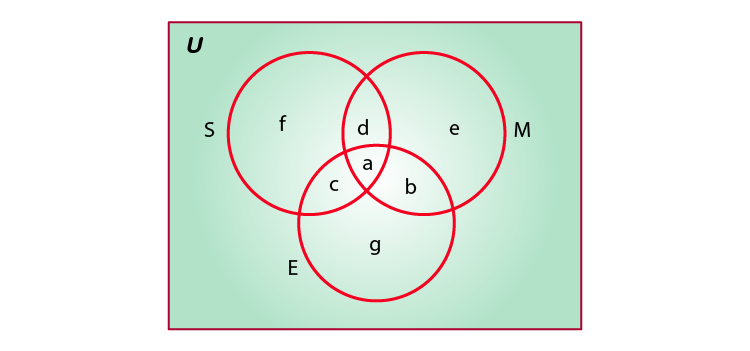n(M ∩ S ∩ E) = a = 4

n(M ∩ S) = a + d = 7

⇒ 4 + d = 7

⇒ d = 3

n(M ∩ E) = a + b = 6

⇒ 4 + b = 6

⇒ b = 2

n(S ∩ E) = a + c = 4

⇒ 4 + c = 4

⇒ c = 0

n(M) = e + d + a + b = 12

⇒ e + 4 + 3 + 2 = 12

⇒ e + 9 = 12

⇒ e = 3

n(E) = g + c + a + b = 15

⇒ g + 0 + 4 + 2 = 15

⇒ g + 6 = 15

⇒ g = 9

n(S) = f + c + a + d = 8

⇒ f + 0 + 4 + 3 = 8

⇒ f + 7 = 8

⇒ f = 1

Therefore, from the above equations, we get that,

(i) Number of students passed in English and Mathematics but not in Science = b = 2

(ii) Number of students in Mathematics and Science but not in English = d = 3

(iii) Number of students in Mathematics only = e = 3

(iv) Number of students in more than one subject only = a + b + c + d = 4 + 3 + 2 + 0 = 9

25. In a class of 60 students, 25 students play cricket and 20 students play tennis, and 10 students play both the games. Find the number of students who play neither?

Solution:

According to the question,

Total number of students = 60

Students who play cricket = 25

Students who play tennis = 20

Students who play both the games = 10

To find: number of students who play neither

Let the total number of students = S

Let the number of students who play cricket = C

Let the number of students who play tennis = T

n(S) = 60, n(C) = 25, n(T) = 20, n(C ∩ T) = 10

So, Number of students who play either of them,

n(C ∪ T) = n(C) + n(T) – n(C ∩ T)

= 25 + 20 – 10

= 35

Hence, Number of student who play neither = Total – n(C ∪ T)

= 60 – 35

= 25

Therefore, there are 25 students who play neither cricket nor tennis.

26. In a survey of 200 students of a school, it was found that 120 study Mathematics, 90 study Physics and 70 study Chemistry, 40 study Mathematics and Physics, 30 study Physics and Chemistry, 50 study Chemistry and Mathematics and 20 none of these subjects. Find the number of students who study all the three subjects.

Solution:

According to the question,

Total number of students = n(U) = 200

Number of students who study Mathematics = n(M) = 120

Number of students who study Physics = n(P) = 90

Number of students who study Chemistry = n(C) = 70

Number of students who study Mathematics and Physics = n(M ∩ P) = 40

Number of students who study Mathematics and Chemistry = n(M ∩ C) = 50

Number of students who study Physics and Chemistry = n(P ∩ C) = 30

Number of students who study none of them = 20

Let the total number of students = U

Let the number of students who study Mathematics = M

Let the number of students who study Physics = P

Let the number of students who study Chemistry = C

number of students who study all the three subjects n(M ∩ P ∩ C)

Number of students who play either of them = n(P ∪ M ∪ C)

n(P ∪ M ∪ C) = Total – none of them

= 200 – 20

= 180 …(i)

Number of students who play either of them = n(P ∪ M ∪ C)

n(P ∪ M ∪ C) = n(C) + n(P) + n(M) – n(M ∩ P) – n(M ∩ C) – n(P ∩ C) + n(P ∩ M ∩ C)

= 120 + 90 + 70 – 40 – 30 – 50 + n(P ∩ M ∩ C)

= 160 + n(P ∩ M ∩ C) …(ii)

From equation (i) and (ii), we get,

160 + n(P ∩ M ∩ C) = 180

⇒ n(P ∩ M ∩ C) = 180 – 160

⇒ n(P ∩ M ∩ C) = 20

Therefore, there are 20 students who study all the three subjects.

27. In a town of 10,000 families it was found that 40% families buy newspaper A, 20% families buy newspaper B, 10% families buy newspaper C, 5% families buy A and B, 3% buy B and C and 4% buy A and C. If 2% families buy all the three newspapers. Find
(a) The number of families which buy newspaper A only.
(b) The number of families which buy none of A, B and C

Solution:

According to the question,

Total number of families = 10,000

Number of families buying newspaper A = n(A) = 40%

Number of families buying newspaper B = n(B) = 20%

Number of families buying newspaper C = n(C) = 10%

Number of families buying newspaper A and B = n(A ∩ B) = 5%

Number of families buying newspaper B and C = n(B ∩ C) = 3%

Number of families buying newspaper A and C = n(A ∩ C) = 4%

Number of families buying all three newspapers = n(A ∩ B ∩ C) = 2%

Let the total number of families = U

Let the number of families buying newspaper A = A

Let the number of families buying newspaper B = B

Let the number of families buying newspaper C = C

(a) Number of families which buy newspaper A only

Percentage of families which buy newspaper A only

= n(A) – n(A ∩ B) – n(A ∩ C) + n(A ∩ B ∩ C)

= 40 – 5 – 4 + 2

= 33%

Number of families which buy newspaper A only

= ((33/100)×10000)

= 3300

Hence, there are 3300 families which buy newspaper A only

(b) Number of families which buy none of A, B and C

Percentage of families which buy either of A, B and C

= n(A ∪ B ∪ C)

= n(A) + n(B) + n(C) – n(A ∩ B) – n(B ∩ C) – n(A ∩ C) + n(A ∩ B ∩ C)

= 40 + 20 + 10 – 5 – 3 – 4 + 2

= 60%

Percentage of families which buy none of A, B and C

= Total percentage – Number of students who play either

= 100% – 60%

= 40%

Number of families which buy none of A, B and C

= ((40/100)×10000)

= 4000

Hence, there are 4000 families which buy none of A, B and C

28. In a group of 50 students, the number of students studying French, English, Sanskrit were found to be as follows:
French = 17, English = 13, Sanskrit = 15
French and English = 09, English and Sanskrit = 4
French and Sanskrit = 5, English, French and Sanskrit = 3. Find the number of students who study
(i) French only
(ii) English only
(iii) Sanskrit only
(iv) English and Sanskrit but not French
(v) French and Sanskrit but not English
(vi) French and English but not Sanskrit
(vii) at least one of the three languages
(viii) none of the three languages

Solution:

According to the question,

Total number of students = 50

Number of students studying English = 13

Number of students studying French = 17

Number of students studying Sanskrit = 15

Number of students studying English and French = 9

Number of students studying French and Sanskrit = 5

Number of students studying English and Sanskrit = 4

Number of students studying all three subjects = 3

Let the total number of students = U

Let the number of students studying English = E

Let the number of students studying French = F

Let the number of students studying Sanskrit = S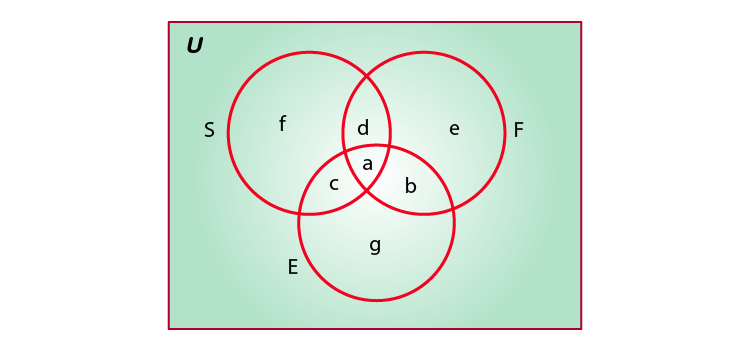n(F ∩ S ∩ E) = a = 3

n(F ∩ S) = a + d = 5

⇒ 3 + d = 5

⇒ d = 2

n(F ∩ E) = a + b = 9

⇒ 3 + b = 9

⇒ b = 6

n(S ∩ E) = a + c = 4

⇒ 3 + c = 4

⇒ c = 1

n(F) = e + d + a + b = 17⇒ e + 2 + 3 + 6 = 17

⇒ e + 11 = 17

⇒ e = 6

n(E) = g + c + a + b = 13

⇒ g + 1 + 3 + 6 = 13

⇒ g + 10 = 13

⇒ g = 3

n(S) = f + c + a + d = 15

⇒ f + 1 + 3 + 2 = 15

⇒ f + 6 = 15

⇒ f = 9

Therefore, from the above equations, we get that,

(i) Number of students studying French only = e = 6

(ii) Number of students studying English only = g = 3

(iii) Number of students studying Sanskrit only = f = 9

(iv) Number of students studying English and Sanskrit but not French = c = 1

(v) Number of students studying French and Sanskrit but not English = d = 2

(vi) Number of students studying French and English but not Sanskrit = b = 6

(vii) Number of students studying at least one of the three languages = a + b + c + d + e + f + g

= 3 + 6 + 1 + 2 + 6 + 9 + 3

= 30

(viii) Number of students studying none of the three languages = Total – (a+b+c+d+e+f+g)

= 50 – (3 + 6 + 1 + 2 + 6 + 9 + 3)

= 50 – 30

= 20

29. Suppose A1, A2, …, A30 are thirty sets each having 5 elements and B1, B2, …, Bn are n sets each with 3 elements, let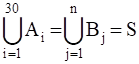and each element of S belongs to exactly 10 of the Ai’s and exactly 9 of the B,’S. then n is equal to
A. 15
B. 3
C. 45
D. 35

Solution:

According to the question,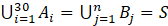Since elements are not repeating, number of elements in A1∪ A2∪ A3∪ ………∪ A30 = 30 × 5

Now, since each element is used 10 times

We get,

10 × S = 30 × 5

⇒ 10 × S = 150

⇒ S = 15

Since elements are not repeating, number of elements in B1∪ B2∪ B3∪ ………∪ Bn = 3 × n

Now, since each element is used 9 times

We get,

9 × S = 3 × n

⇒ 9 × S = 3n

⇒ S = n/3

⇒ n/3 = 15

⇒ n = 45

Therefore, the value of n is 45

Hence, Option (C) 45, is the correct answer.